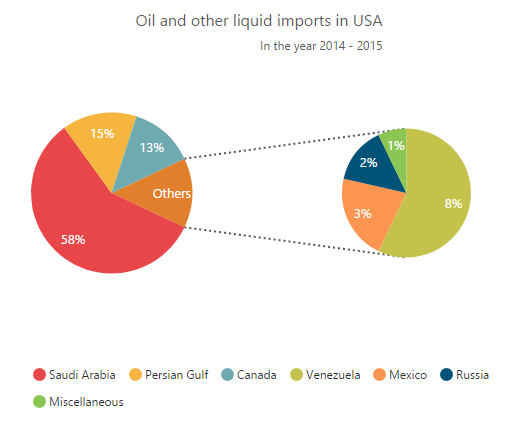# Line Chart in ASP.NET MVC Chart

13 Jun 202324 minutes to read

To render a Line Chart, set the series Type as Line in the chart series. To change the line segment color, you can use the Fill property of the series.

• CSHTML
• ``````@(Html.EJ().Chart("chartContainer")

// ...

.Series(sr =>
{
//Change type and color of the series.
})

//...
)``````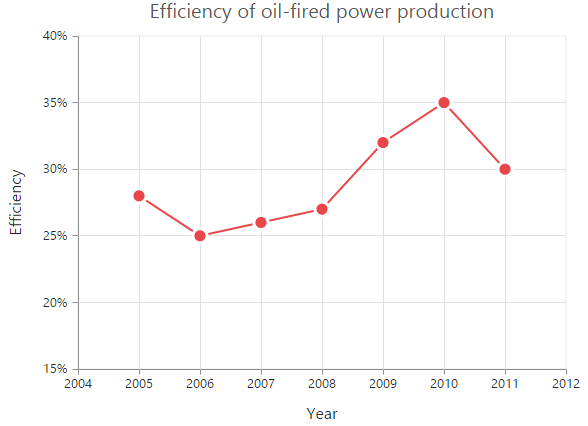### Change the line width

To change the width of the line segment, you can use the Width property in the series.

• CSHTML
• ``````@(Html.EJ().Chart("chartContainer")

// ...

.Series(sr =>
{
//Change the width of line series
})

//...
)``````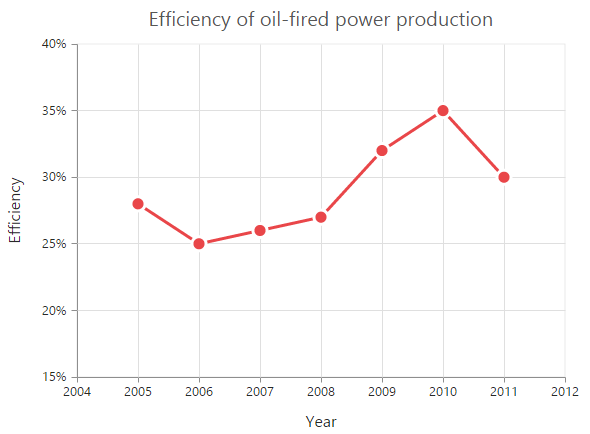### Dashed lines

To render the line series with dotted lines, you can use the DashArray option of the series.

• CSHTML
• ``````@(Html.EJ().Chart("chartContainer")

// ...

.Series(sr =>
{
//Change dash array to display dotted or dashed lines
})

//...
)``````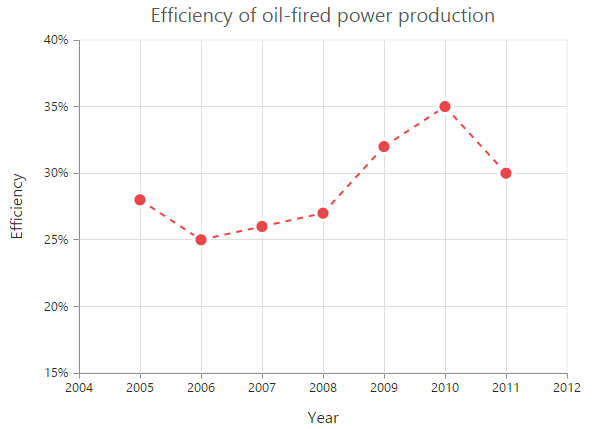### Changing the line cap

For customizing the start and end caps of the line segment, you can use the LineCap property.

• CSHTML
• ``````@(Html.EJ().Chart("chartContainer")

// ...

.Series(sr =>
{
//Change line cap
})

//...
)``````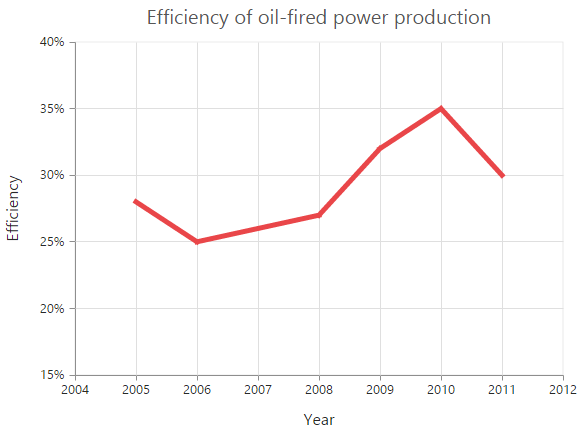### Changing the line join

You can use the LineJoin property to specify how two intersecting line segments should be joined.

• CSHTML
• ``````@(Html.EJ().Chart("chartContainer")

// ...

.Series(sr =>
{
//Change line join
})

//...
)``````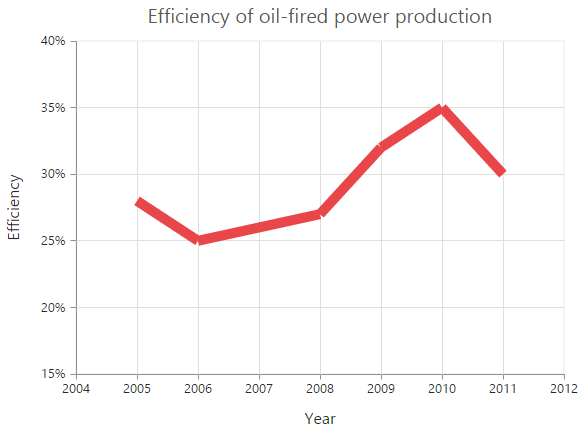### MultiColor Line

You can change the color of the line segments by using the Fill property of the each Points in the series.

• CSHTML
• ``````@(Html.EJ().Chart("chartContainer")
//...
.Series(sr =>
{
sr.Points(pt=>{
})
//...
)``````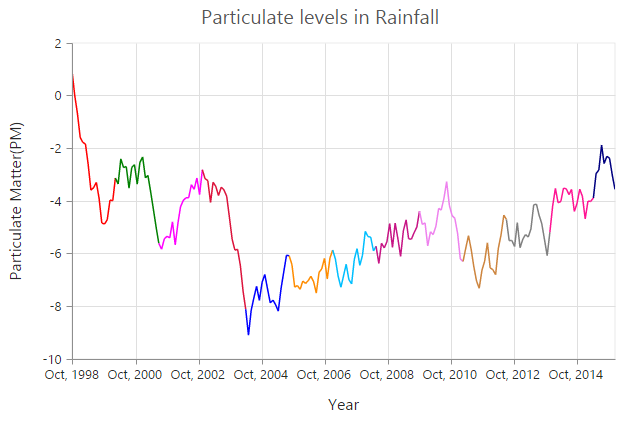## Step Line Chart

To render a Step Line Chart, set the series Type as StepLine” in the chart series. To change the StepLine segment color, you can use the Fill property of the series.

• CSHTML
• ``````@(Html.EJ().Chart("chartContainer")

// ...

.Series(sr =>
{
//Change type and color of the series.
})

//...
)``````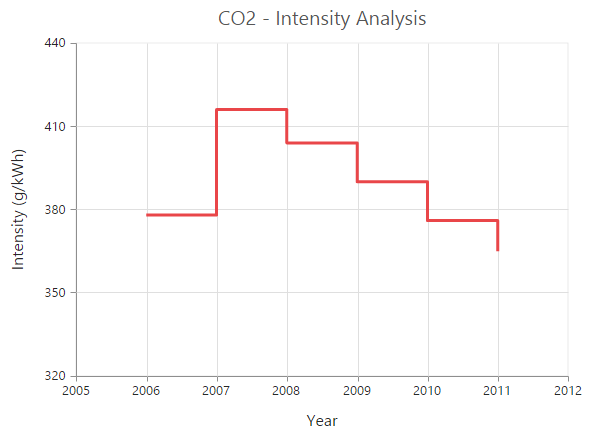### Changing the line width

To change the line width, you can use the Width property.

• CSHTML
• ``````@(Html.EJ().Chart("chartContainer")

// ...

.Series(sr =>
{
//Change the width of step line series
})

//...
)``````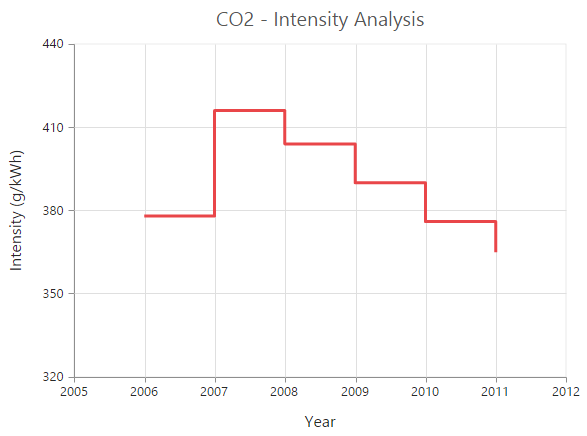### Dashed lines

To render the step line series with dotted lines, you can use the DashArray option of the series.

• CSHTML
• ``````@(Html.EJ().Chart("chartContainer")

// ...

.Series(sr =>
{
//Change dash array to display dotted or dashed lines
})

//...
)``````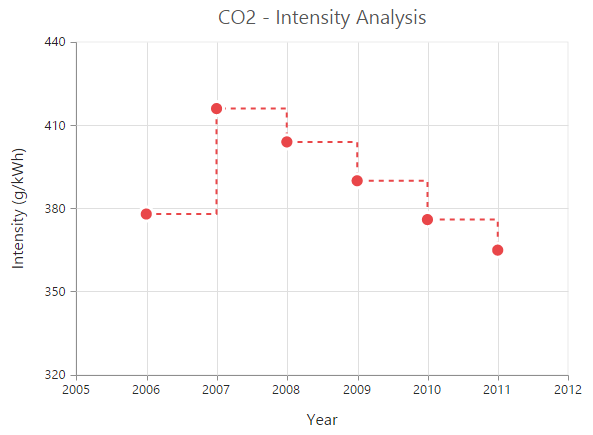### Changing the line cap

For customizing the start and end caps of the line segment, you can use the LineCap property.

• CSHTML
• ``````@(Html.EJ().Chart("chartContainer")

// ...

.Series(sr =>
{
//Change line cap
})

//...
)``````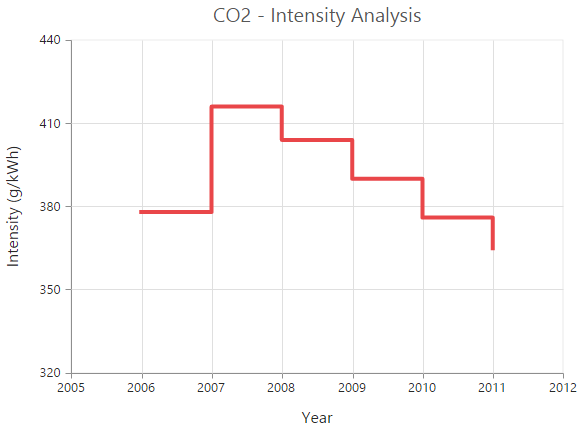### Changing the line join

You can use the LineJoin property to specify how two intersecting line segments should be joined.

• CSHTML
• ``````@(Html.EJ().Chart("chartContainer")

// ...

.Series(sr =>
{
//Change line join
})

//...
)``````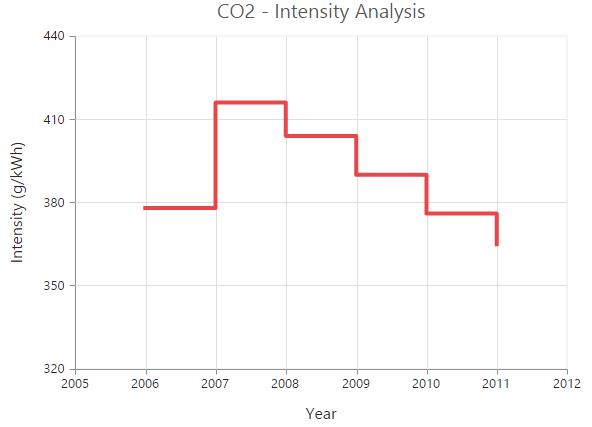## Area Chart

To render an Area chart, you can specify the series Type as Area in the chart series. To change the Area color, you can use the Fill property of the series.

• CSHTML
• ``````@(Html.EJ().Chart("chartContainer")

// ...

.Series(sr =>
{
//Change type and color of the series.
})

//...
)``````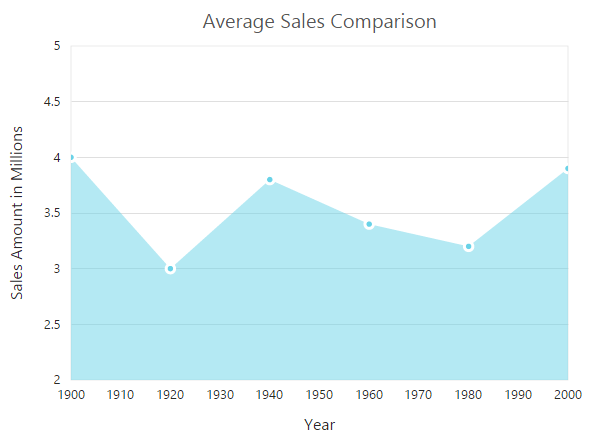## Range Area Chart

To render a Range Area Chart, set the Type as RangeArea in the chart series. To change the RangeArea color, you can use the Fill property of the series.

Since the RangeArea series requires two y values for a point, you have to add the High and Low value. High and Low value specifies the maximum and minimum range of the points.

• When you are using the Points option, specify the high and low values by using the High and Low option of the point.

• When you are using the DataSource option to assign the data, map the fields from the dataSource that contain High and Low values by using the Series.High and Series.Low options.

• CSHTML
• ``````@(Html.EJ().Chart("chartContainer")

// ...

.Series(sr =>
{
//Change the series type and fill color
sr.Type(SeriesType.RangeArea).Fill("Indigo")
//Use High and Low values instead of Y
//..
})
})

//...
)``````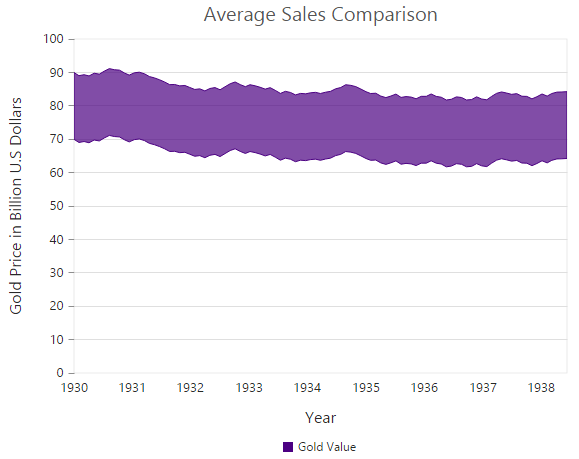## Step Area Chart

To render a Step Area Chart, set the Type as StepArea in the chart series. To change the StepArea color, you can use the Fill property of the series.

• CSHTML
• ``````@(Html.EJ().Chart("chartContainer")

// ...

.Series(sr =>
{
//Change the series type and fill color
sr.Type(SeriesType.StepArea).Fill("#69D2E7")
//...
})

//...
)``````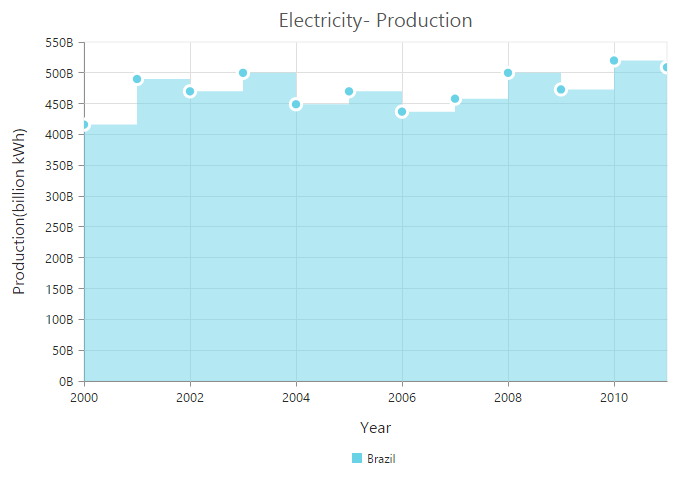## Spline Area Chart

To render a Spline Area Chart, set the Type as SplineArea in the chart series. To change the SplineArea color, you can use the Fill property of the series.

• CSHTML
• ``````@(Html.EJ().Chart("chartContainer")

// ...

.Series(sr =>
{
//Change the series type and fill color
sr.Type(SeriesType.SplineArea).Fill("#C4C24A")
//...
})

//...
)``````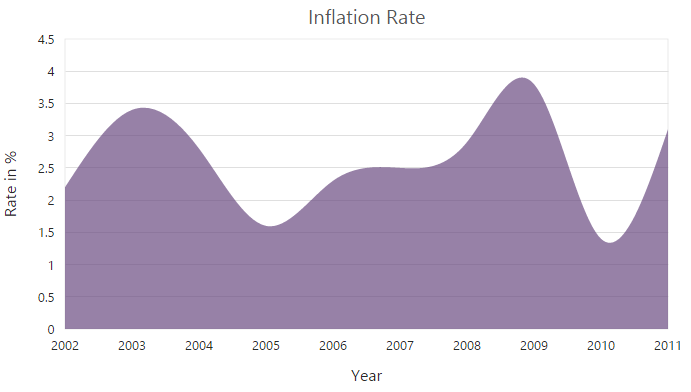## Stacked Area Chart

To render a Stacked Area Chart, set the Type as StackingArea in the chart series. To change the StackingArea color, you can use the Fill property of the series.

• CSHTML
• ``````@(Html.EJ().Chart("chartContainer")

// ...

.Series(sr =>
{
//Change the series type and fill color
sr.Type(SeriesType.StackingArea).Fill("#69D2E7")
//...
})

//...
)``````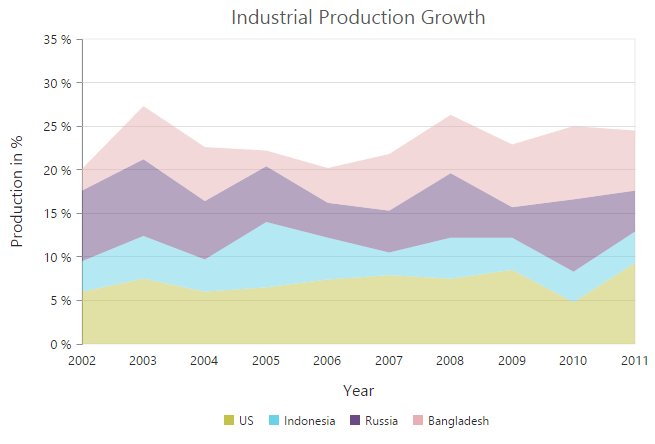## 100% Stacked Area Chart

To render a 100% Stacked Area Chart, set the Type as StackingArea100 in the chart series. To change the StackingArea100 color, you can use the Fill property of the series.

• CSHTML
• ``````@(Html.EJ().Chart("chartContainer")

// ...

.Series(sr =>
{
//Change the series type and fill color
sr.Type(SeriesType.StackingArea100).Fill("#C4C24A")
//...
})

//...
)``````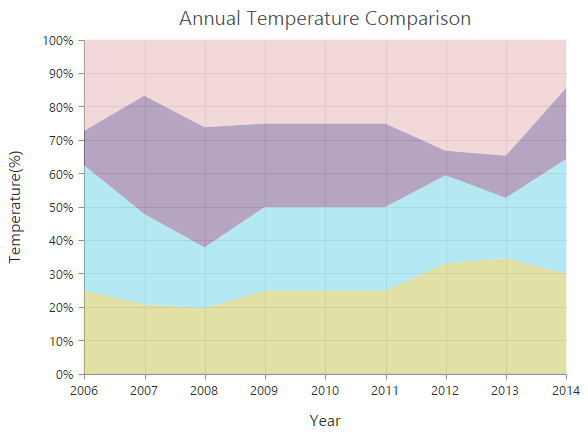## Column Chart

To render a Column Chart, set the Type as Column in the chart series. To change the color of the column series, you can use the Fill property.

• CSHTML
• ``````@(Html.EJ().Chart("chartContainer")

// ...

.Series(sr =>
{
//Change the series type and fill color
sr.Type(SeriesType.Column).Fill("#E94649")
//...
})

//...
)``````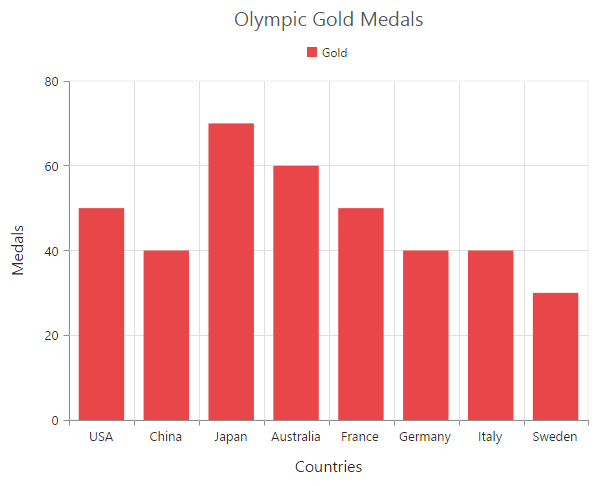### Change a point color

You can change the color of a column by using the Fill property of the point.

• CSHTML
• ``````@(Html.EJ().Chart("chartContainer")

// ...

.Series(sr =>
{
//...
})

//...
)``````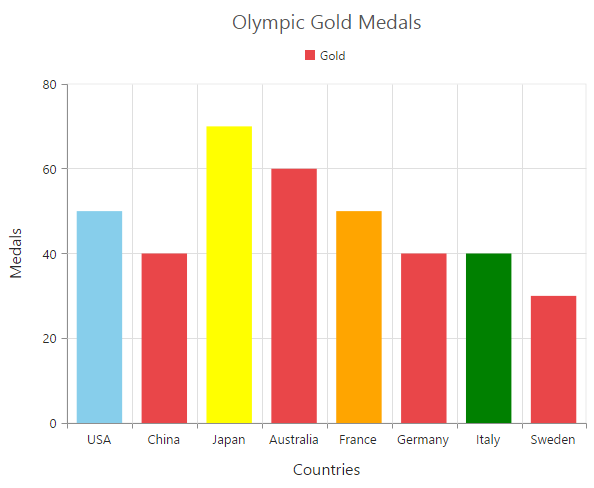### Column width customization

Width of any column type series can be customized by using the ColumnWidth property. Default value of ColumnWidth is 0.7. Value ranges from 0 to 1. Here 1 corresponds to 100% of available width and 0 corresponds to 0% of available width.

NOTE

Width of a column also depends upon the ColumnSpacing property, because ColumnSpacing will reduce the space available for drawing a column. This is also applicable for StackingColumn, StackingColumn100, Bar, StackingBar, StackingBar100, RangeColumn, HiLo, HiLoOpenClose, Candle and Waterfall charts.

• CSHTML
• ``````@(Html.EJ().Chart("chartContainer")

.Series(sr =>
{
//Customizing column width for a specific series
//...
})
// ...
)``````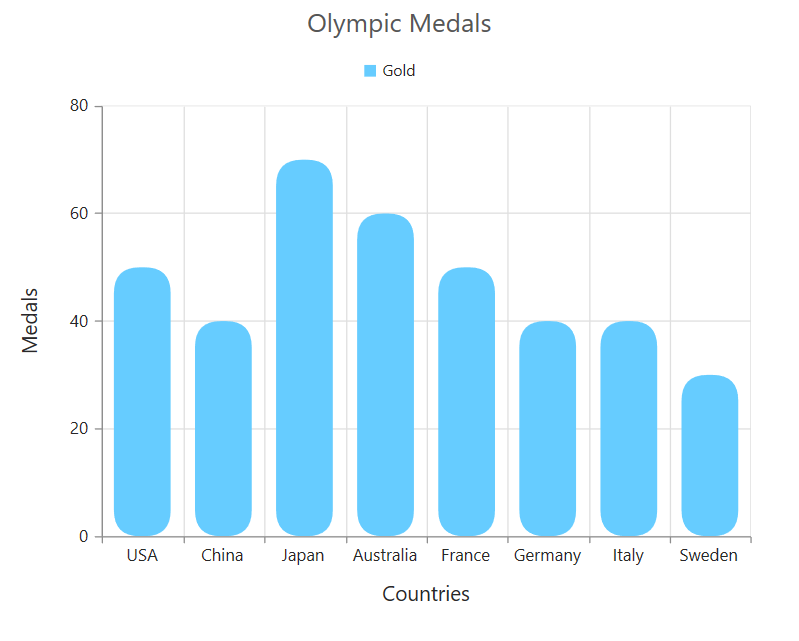### Column with rounded corners

Corners of the column chart can be customized by setting value to the CornerRadius property.

• CSHTML
• ``````@(Html.EJ().Chart("container")
))``````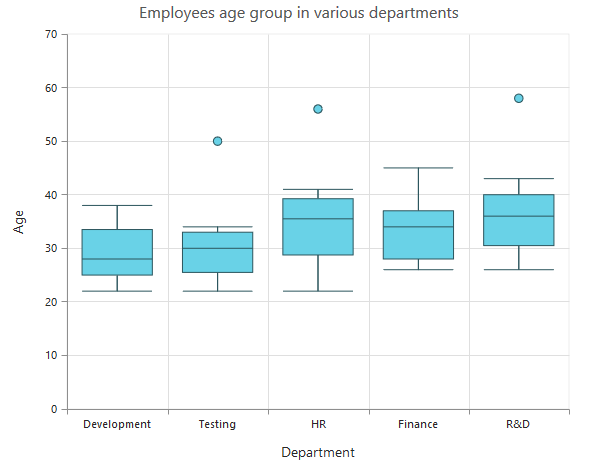### Spacing between column series

Spacing between column type series can be customized using the ColumnSpacing property. Default value of ColumnSpacing is 0. Value ranges from 0 to 1. Here 1 corresponds to 100% available space and 0 corresponds to 0% available space.

NOTE

Space between columns will also affect the width of the column. For example, setting 20% spacing and 100% width will render columns with 80% of total width. This is also applicable for StackingColumn, StackingColumn100, Bar, StackingBar, StackingBar100, RangeColumn, HiLo, HiLoOpenClose, Candle and Waterfall charts.

• CSHTML
• ``````@(Html.EJ().Chart("chartContainer")

.Series(sr =>
{
//Customizing column spacing for a specific series
//...
})
// ...
)``````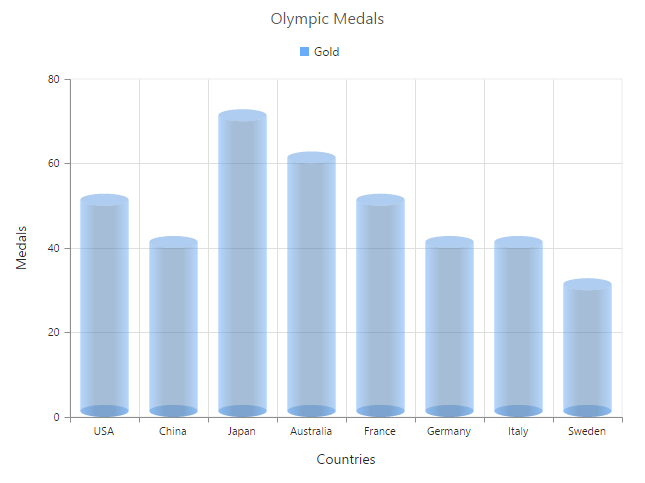### Cylindrical Chart

To render a cylindrical chart, set the ColumnFacet property as “Cylinder” in the chart series along with the series type.

The following chart types can be rendered as cylinder in both 2D and in 3D view.

• Column Chart, Bar Chart, Stacked Column Chart, Stacked Bar Chart, 100% Stacked Column Chart, 100% Stacked Bar Chart.
• CSHTML
• ``````@(Html.EJ().Chart("chartContainer")

// ...

.Series(sr =>
{
// To change the shape of the series.
})

//...
)``````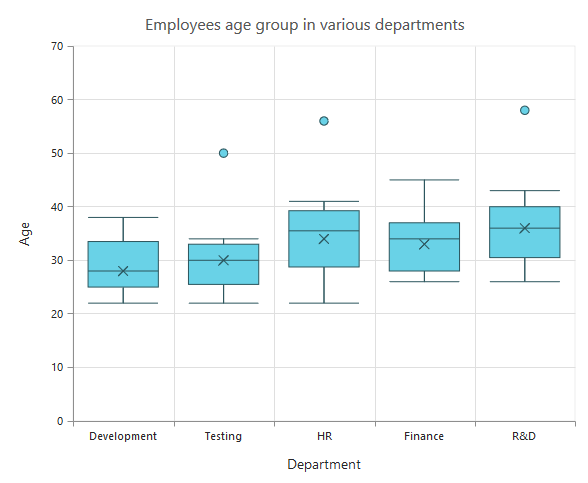## RangeColumn Chart

To render a Range Column Chart, set the Type as RangeColumn in the chart series. To change the RangeColumn color, use the Fill property of the series.

Since, the RangeColumn series requires two y values for a point, add the High and Low value. High and Low value specifies the maximum and minimum range of the points.

• When you are using the Points option, specify the high and low values by using the High and Low option of the point.

• When you are using the DataSource option to assign the data, you have to map the fields from the dataSource that contains high and low values by using the Series.High and Series.Low) options.

• CSHTML
• ``````@(Html.EJ().Chart("chartContainer")

// ...

.Series(sr =>
{
//Set chart type to series
sr.Type(SeriesType.RangeColumn).Fill("#E94649")
//Use high and low values instead of y
//...
})

//...
)``````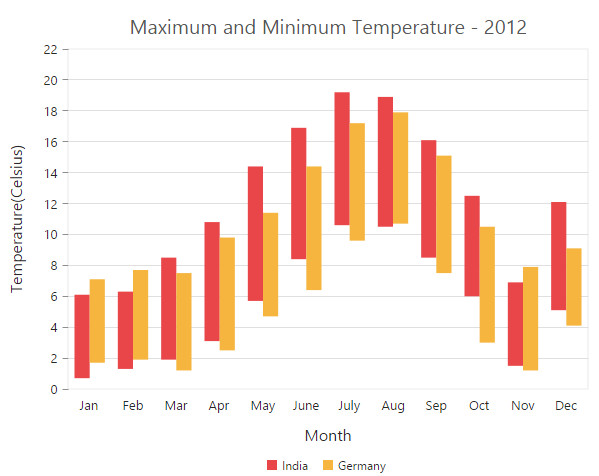### Change a point color

To change the color of a range column, you can use the Fill property of point.

• CSHTML
• ``````@(Html.EJ().Chart("chartContainer")

// ...

.Series(sr =>
{
//change a point color of Range column
//...
})

//...
)``````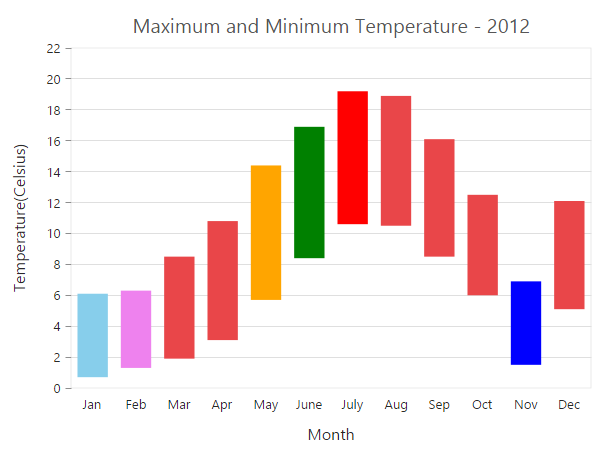## Stacked Column Chart

To render a Stacked Column Chart, set the Type as StackingColumn in the chart series. To change the StackingColumn color, you can use the Fill property of the series.

• CSHTML
• ``````@(Html.EJ().Chart("chartContainer")

// ...

.Series(sr =>
{
//Set chart type to series
sr.Type(SeriesType.StackingColumn).Fill("#E94649")
//...
})

//...
)``````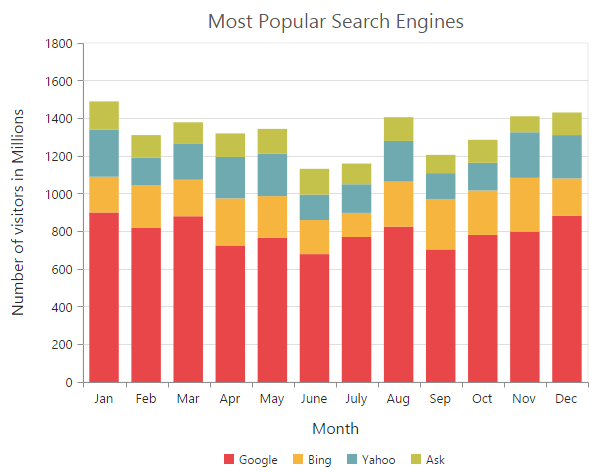### Cluster / Group stacked columns

You can use the StackingGroup property to group the stacked columns. Columns with same group name are stacked on top of each other.

• CSHTML
• ``````@(Html.EJ().Chart("chartContainer")

// ...

.Series(sr =>
{
// For grouping stacked columns
sr.StackingGroup("GroupOne")
//...
// For grouping stacked columns
sr.StackingGroup("GroupOne")
//...
})

//...
)``````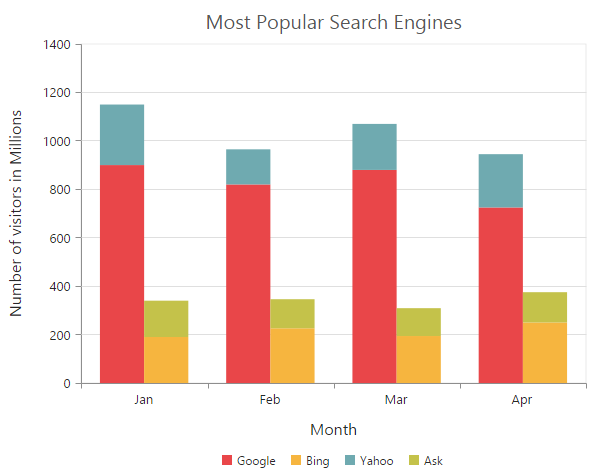### Change a point color

To change the color of a stacking column, you can use the Fill property of the point.

• CSHTML
• ``````@(Html.EJ().Chart("chartContainer")

// ...

.Series(sr =>
{
//change a point color of Stacking column
//...
})

//...
)``````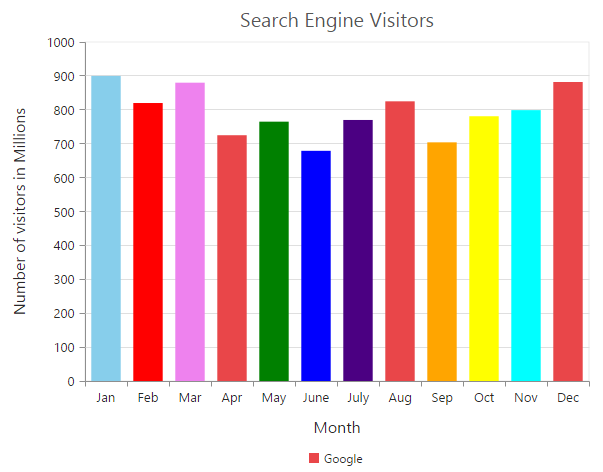## 100% Stacked Column Chart

To render a 100% Stacked Column Chart, set the Type as StackingColumn100 in the chart series. To change the StackingColumn100 color, you can use the Fill property of the series.

• CSHTML
• ``````@(Html.EJ().Chart("chartContainer")

// ...

.Series(sr =>
{
//Change type and color of the series
sr.Type(SeriesType.StackingColumn100).Fill("#E94649")
//...
})

//...
)``````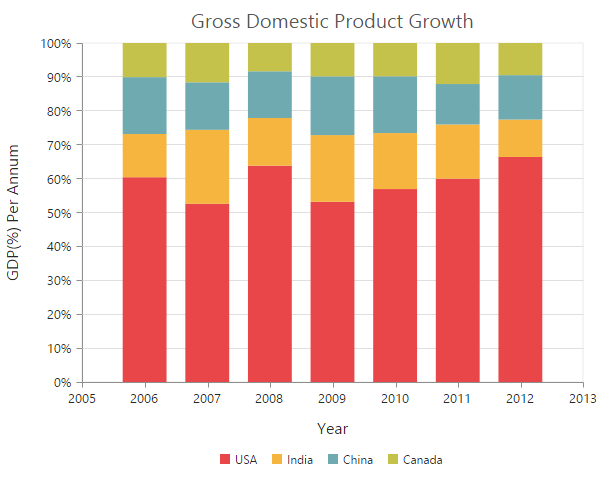### Cluster / Group 100% stacked columns

By using the StackingGroup property, you can group the 100% stacking columns. Columns with same group name are stacked on top of each other.

• CSHTML
• ``````@(Html.EJ().Chart("chartContainer")

// ...

.Series(sr =>
{
// For grouping 100% stacked columns
sr.StackingGroup("GroupOne")
//...
// For grouping 100% stacked columns
sr.StackingGroup("GroupOne")
//...
})

//...
)``````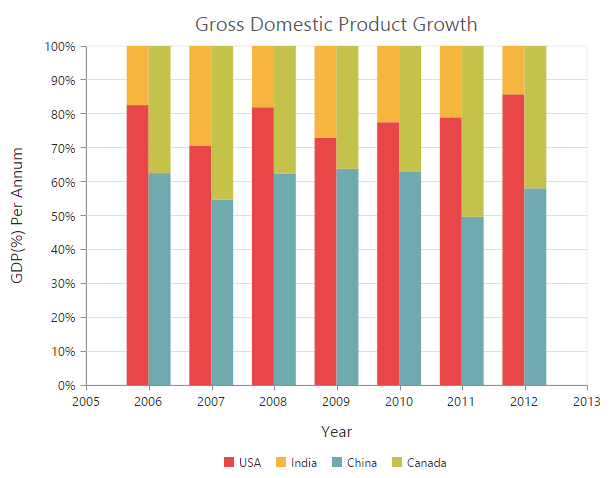### Change a point color

To change the color of a 100% stacking column, you can use the Fill property of the point.

• CSHTML
• ``````@(Html.EJ().Chart("chartContainer")

// ...

.Series(sr =>
{
//change a point color of 100% Stacking column
//...
})

//...
)``````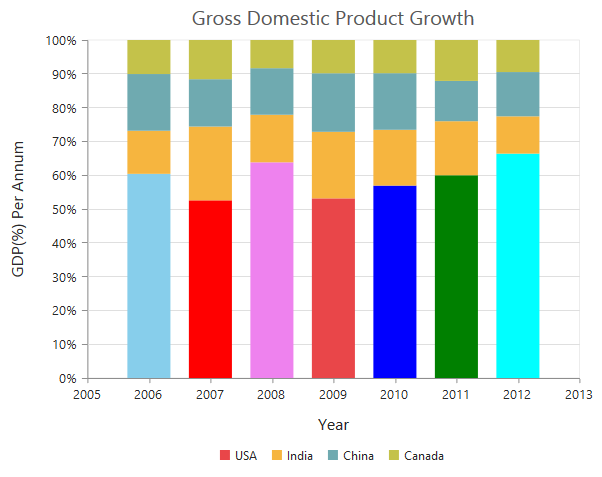## Bar Chart

To render a bar Chart, set the Type as Bar in the chart series. To change the bar color, you can use the Fill property of the series.

• CSHTML
• ``````@(Html.EJ().Chart("chartContainer")

// ...

.Series(sr =>
{
//Change type and color of the series
sr.Type(SeriesType.Bar).Fill("#E94649")
//...
})

//...
)``````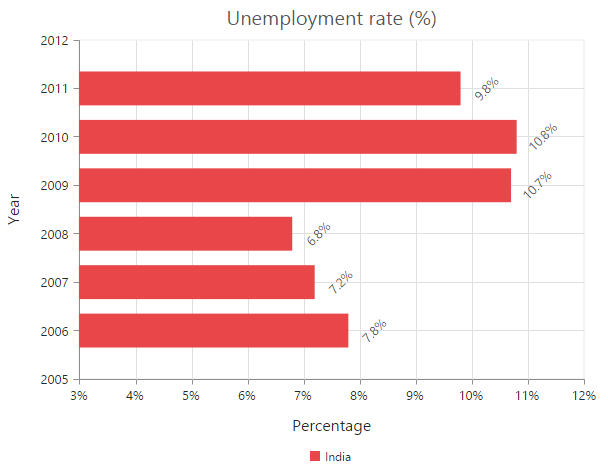### Change the color of a bar

By using the Fill property of the point, you can change the specific point of the series.

• CSHTML
• ``````@(Html.EJ().Chart("chartContainer")

// ...

.Series(sr =>
{
//change a point color of bar
//...
})

//...
)``````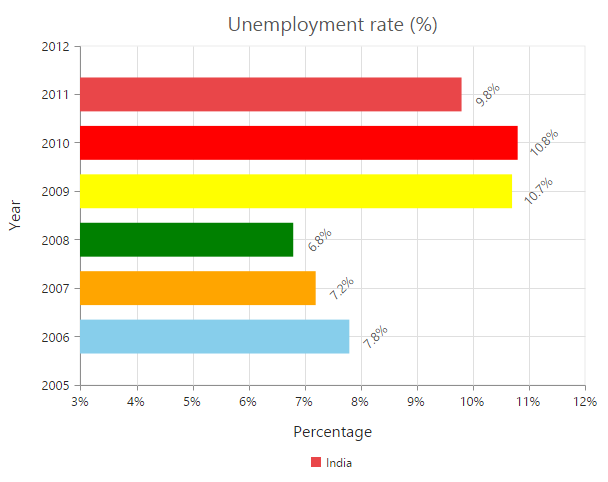## Stacked Bar Chart

To render a Stacked Bar Chart, set the Type as StackingBar in the chart series. To change the StackingBar color, you can use the Fill property of the series.

• CSHTML
• ``````@(Html.EJ().Chart("chartContainer")

// ...

.Series(sr =>
{
//Change type and color of the series
sr.Type(SeriesType.StackingBar).Fill("#E94649")
//...
})

//...
)``````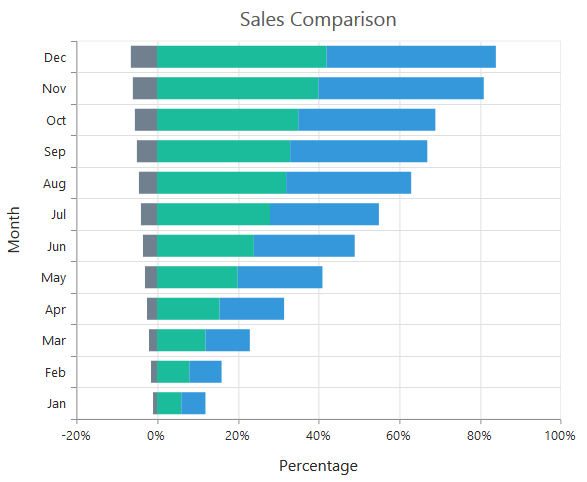### Cluster / Group stacked bars

You can use the StackingGroup property to group the stacking bars with the same group name.

• CSHTML
• ``````@(Html.EJ().Chart("chartContainer")

// ...

.Series(sr =>
{
// For grouping stacking bar
sr.StackingGroup("GroupOne")
//...
// For grouping stacking bar
sr.StackingGroup("GroupOne")
//...
})

//...
)``````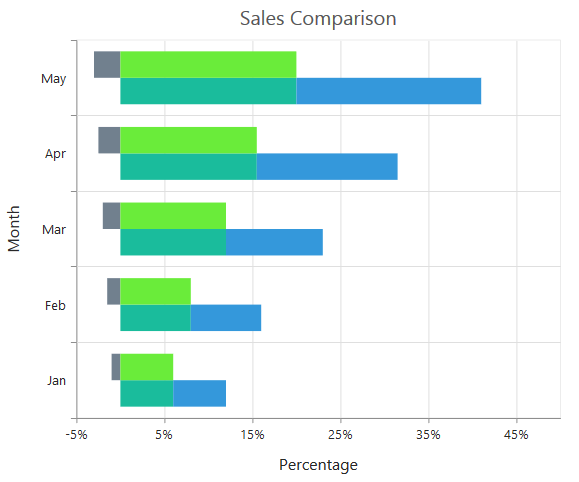### Change a point color

You can change the color of a stacking bar by using the Fill property of the point.

• CSHTML
• ``````@(Html.EJ().Chart("chartContainer")

// ...

.Series(sr =>
{
//change a point color of stacking bar
//...
})

//...
)``````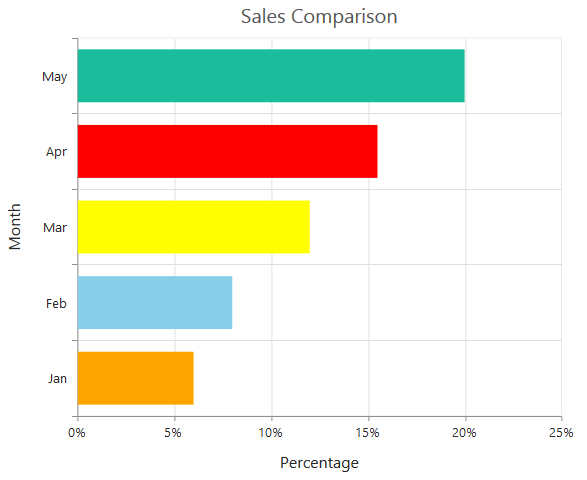## 100% Stacked Bar Chart

To render a 100% Stacked Bar Chart, set the Type as StackingBar100 in the chart series. To change the StackingBar100 color, you can use the Fill property of the series.

• CSHTML
• ``````@(Html.EJ().Chart("chartContainer")

// ...

.Series(sr =>
{
//Change type and color of the series
sr.Type(SeriesType.StackingBar100).Fill("#E94649")
//...
})

//...
)``````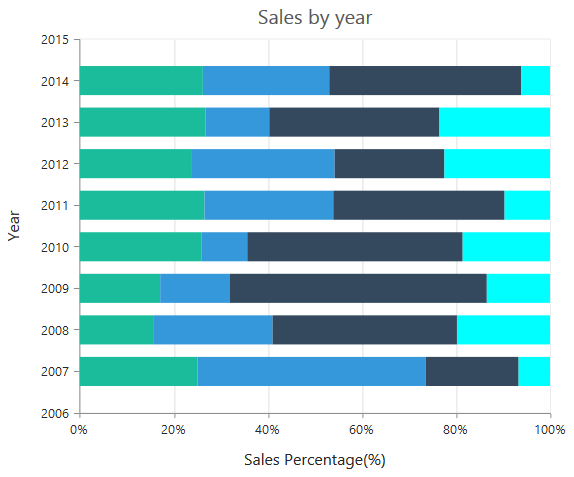By using the StackingGroup property, you can group the 100% stacking bars with the same group name.

• CSHTML
• ``````@(Html.EJ().Chart("chartContainer")

// ...

.Series(sr =>
{
// For grouping 100% stacking bar
sr.StackingGroup("GroupOne")
//...
// For grouping 100% stacking bar
sr.StackingGroup("GroupOne")
//...
})

//...
)``````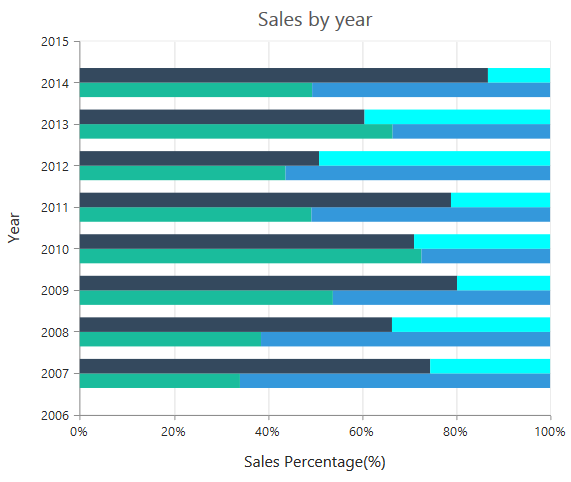### Change a point color

To change the color of a 100% stacking bar, you can use the Fill property of the point.

• CSHTML
• ``````@(Html.EJ().Chart("chartContainer")

// ...

.Series(sr =>
{
//change a point color of 100% stacking bar
//...
})

//...
)``````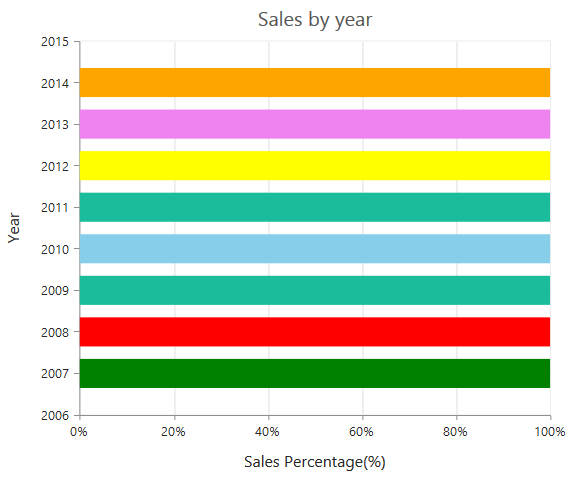## Spline Chart

To render a Spline Chart, set the Type as Spline in the chart series. To change the Spline segment color, you can use the Fill property of the series.

• CSHTML
• ``````@(Html.EJ().Chart("chartContainer")

// ...

.Series(sr =>
{
//Change type and color of the series
//...
})

//...
)``````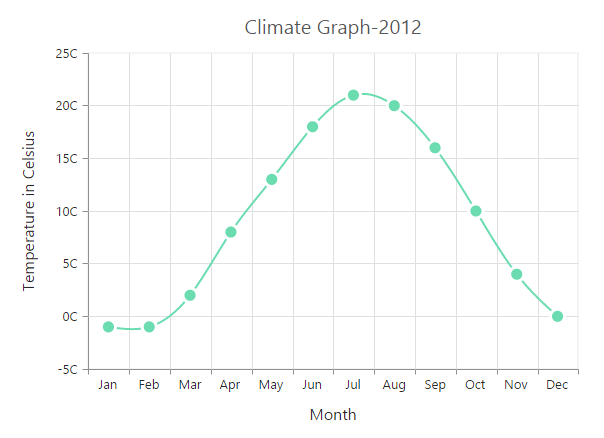### Change the spline width

To change the spline segment width, you can use the Width property of the series.

• CSHTML
• ``````@(Html.EJ().Chart("chartContainer")

// ...

.Series(sr =>
{
//Change the width of spline series
sr.Width(3)
//...
})

//...
)``````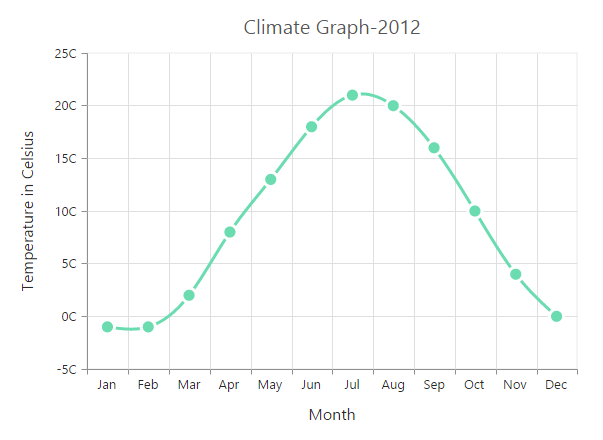### Dashed lines

To render the spline series with dotted lines, you can use the DashArray option of the series.

• CSHTML
• ``````@(Html.EJ().Chart("chartContainer")

// ...

.Series(sr =>
{
//Change dash array to display dotted or dashed splines
sr.DashArray("5,5")
//...
})

//...
)``````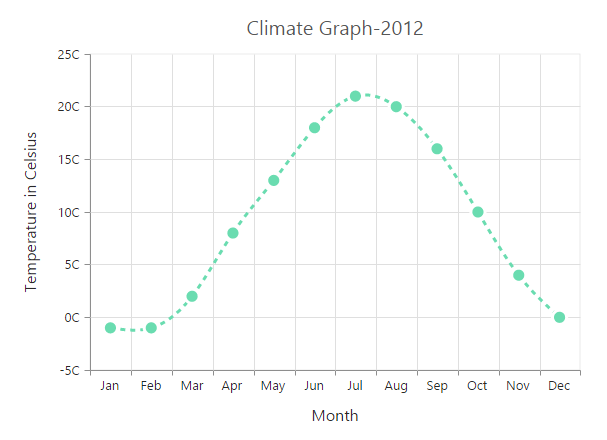## Pie Chart

You can create a pie chart by setting the series Type as Pie in the chart series.

• CSHTML
• ``````@(Html.EJ().Chart("chartContainer")

// ...

.Series(sr =>
{
//Set chart type to series
sr.Type(SeriesType.Pie)
//...
})

//...
)``````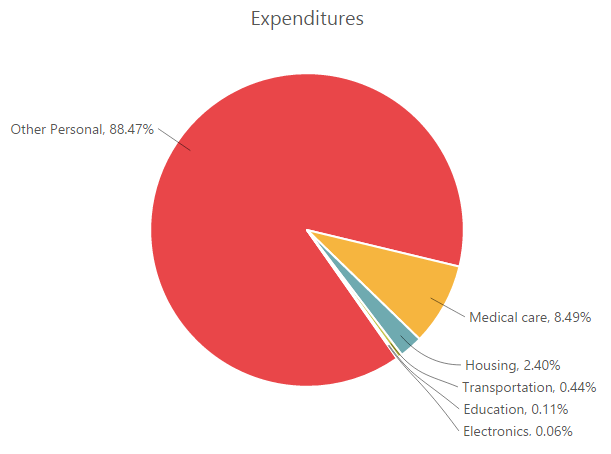### Change the pie size

You can use the PieCoefficient property to change the diameter of the Pie chart with respect to the plot area. It ranges from 0 to 1 and the default value is 0.8.

• CSHTML
• ``````@(Html.EJ().Chart("chartContainer")

// ...

.Series(sr =>
{
//Change pie chart coefficient value
sr.PieCoefficient(0.4f)
//...
})

//...
)``````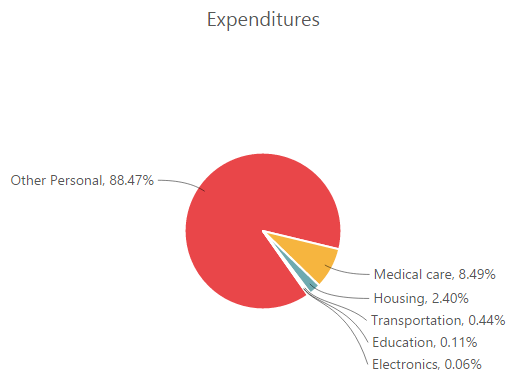### Explode a pie segment

You can explode a pie segment on the chart load by using the ExplodeIndex of the series.

• CSHTML
• ``````@(Html.EJ().Chart("chartContainer")

// ...

.Series(sr =>
{
//Set point index value to explode the pie segment.
sr.ExplodeIndex(1)
//...
})

//...
)``````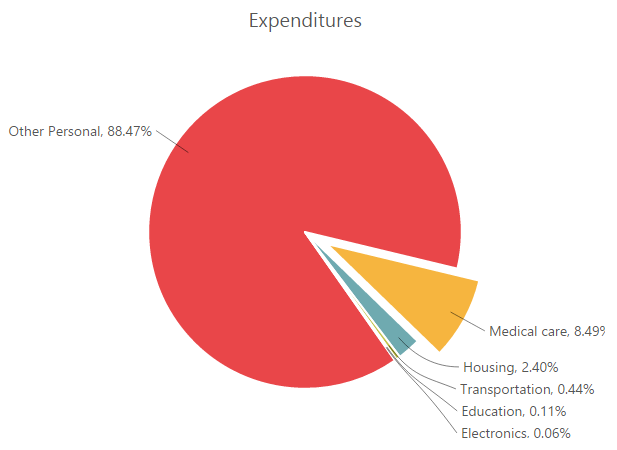### Explode all the segments

To explode all the segments of the Pie chart, you can enable the ExplodeAll property.

• CSHTML
• ``````@(Html.EJ().Chart("chartContainer")

// ...

.Series(sr =>
{
//Enable explodeAll property for pie chart.
sr.ExplodeAll(true)
//...
})

//...
)``````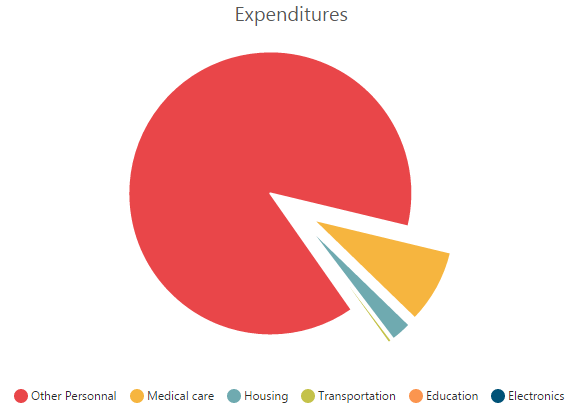### Explode a pie segment on mouse over

To explode a pie segment on a mouse over, you can enable the Explode property of the series.

• CSHTML
• ``````@(Html.EJ().Chart("chartContainer")

// ...

.Series(sr =>
{
//Enable pie explode option on mouse over the chart
sr.Explode(true)
//...
})

//...
)``````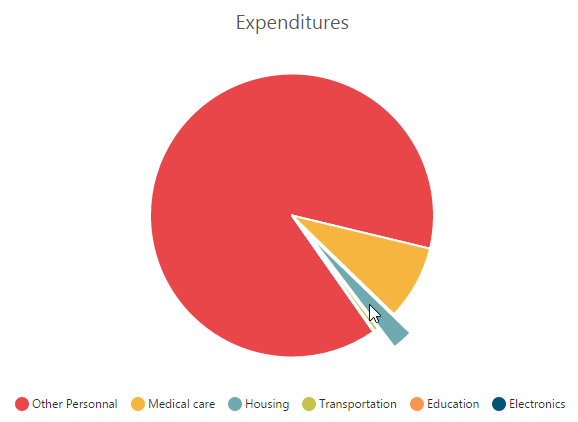### Sector of Pie

EjChart allows you to render all the data points/segments in the semi-pie, quarter-pie or in any sector by using the StartAngle and EndAngle options.

• CSHTML
• ``````@(Html.EJ().Chart("chartContainer")

// ...

.Series(sr =>
{
sr.Type(SeriesType.Pie)
//Set startAngle and endAngle to draw the semi pie chart
.StartAngle(-90).EndAngle(90)
//...
})

//...
)``````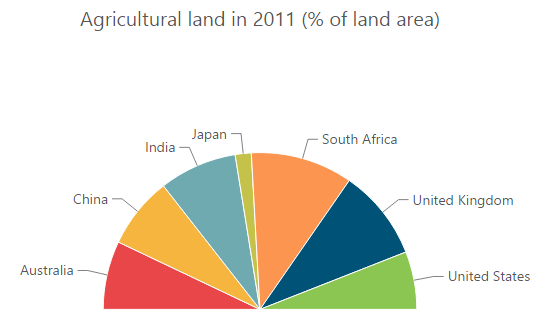## Doughnut Chart

To create a Doughnut chart, you can specify the series Type as Doughnut in the chart series.

• CSHTML
• ``````@(Html.EJ().Chart("chartContainer")

// ...

.Series(sr =>
{
//Set chart type to series
sr.Type(SeriesType.Doughnut)
//...
})

//...
)``````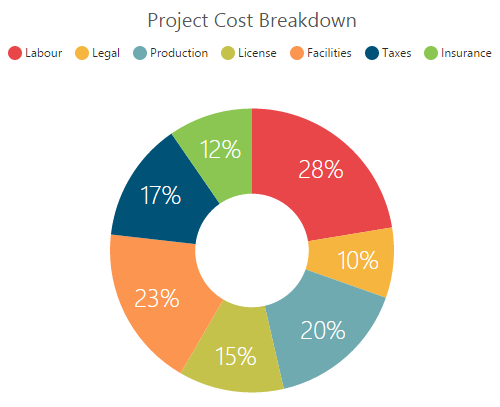You can change the doughnut chart inner radius by using the DoughnutCoefficient with respect to the plot area. It ranges from 0 to 1 and the default value is 0.4.

• CSHTML
• ``````@(Html.EJ().Chart("chartContainer")

// ...

.Series(sr =>
{
//Change doughnut chart coefficient value
sr.DoughnutCoefficient(0.6f)
//...
})

//...
)``````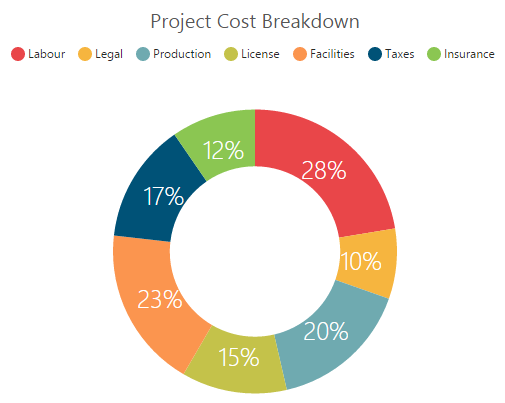### Change the doughnut size

You can use the DoughnutSize property to change the diameter of the Doughnut chart with respect to the plot area. It ranges from 0 to 1 and the default value is 0.8.

• CSHTML
• ``````@(Html.EJ().Chart("chartContainer")

// ...

.Series(sr =>
{
//Change doughnut chart coefficient value
sr.DoughnutSize(0.4f)
//...
})

//...
)``````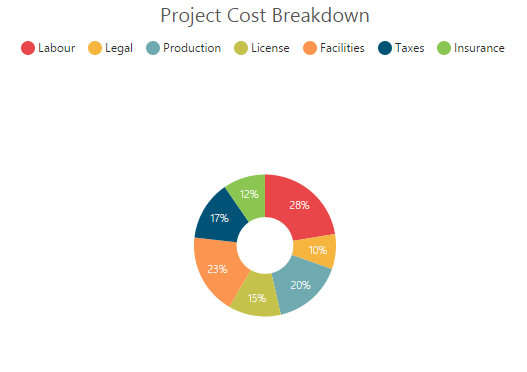### Explode a doughnut segment

To explode a specific doughnut segment, set the index to be exploded by using the ExplodeIndex option of the series.

• CSHTML
• ``````@(Html.EJ().Chart("chartContainer")

// ...

.Series(sr =>
{
//Set point index value to explode the doughnut segment.
sr.ExplodeIndex(1)
//...
})

//...
)``````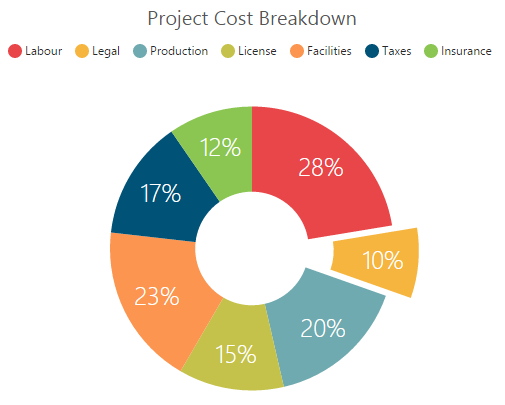### Explode all the segments

To explode all the segments, you can enable the ExplodeAll property of the series.

• CSHTML
• ``````@(Html.EJ().Chart("chartContainer")

// ...

.Series(sr =>
{
//Enable explodeAll property of doughnut chart
sr.ExplodeAll(true)
//...
})

//...
)``````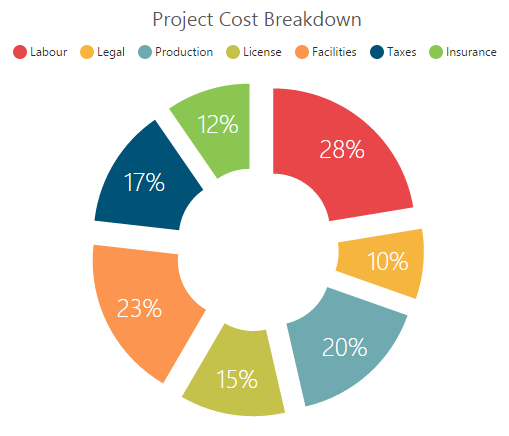### Explode a doughnut segment on mouse over

To explode a doughnut segment on a mouse over, you can enable the Explode property of the series.

• CSHTML
• ``````@(Html.EJ().Chart("chartContainer")

// ...

.Series(sr =>
{
//Enable doughnut explode option on mouse over the chart
sr.Explode(true)
//...
})

//...
)``````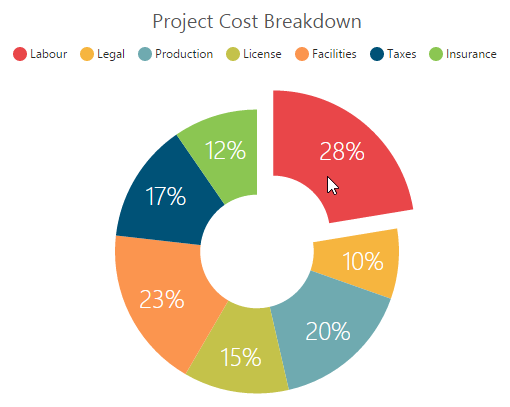### Sector of Doughnut

EjChart allows you to render all the data points/segments in the semi-doughnut, quarter- doughnut or in any sector by using the StartAngle and EndAngle options.

• CSHTML
• ``````@(Html.EJ().Chart("chartContainer")

// ...

.Series(sr =>
{
sr.Type(SeriesType.Doughnut)
//Set startAngle and endAngle to draw the semi doughnut chart
.StartAngle(-90).EndAngle(90)
//...
})

//...
)``````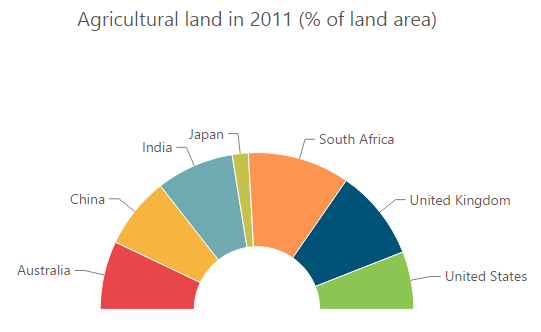## Multiple Pie Chart

EjChart provides support to render more than one series in pie and in doughnut chart. Radius of each series is calculated based on the radius of the previous series. And in addition legend is displayed according to the list of chart series.

• CSHTML
• ``````@(Html.EJ().Chart("chartContainer")

// ...
.Series(sr =>
{
sr.Type(SeriesType.Pie)
//...
})

//...
)``````

Multiple Pie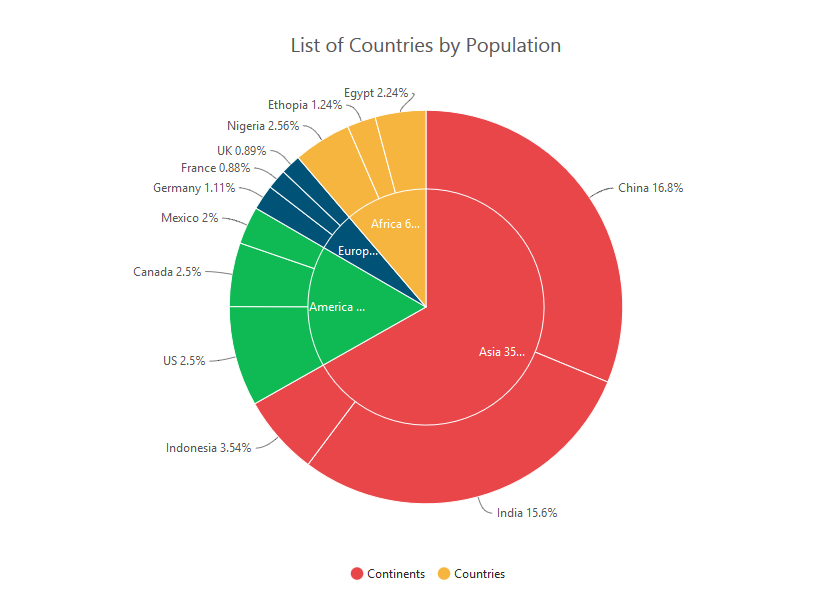Multiple Doughnut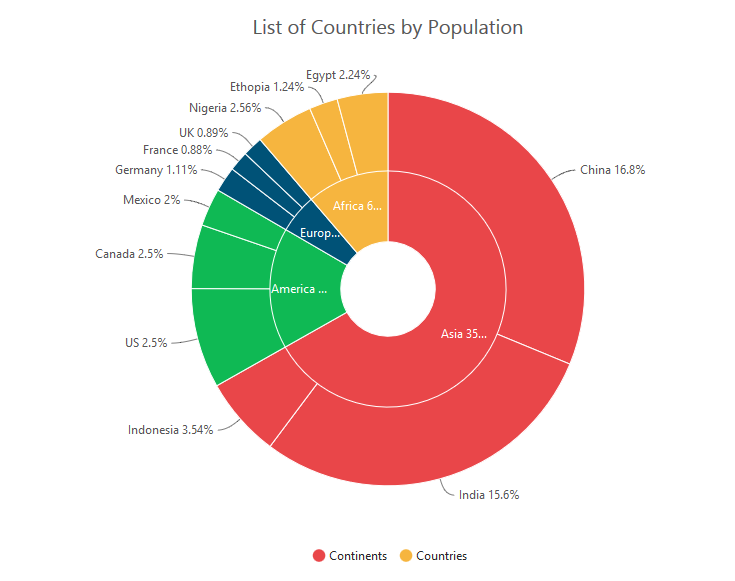### Start and End Angle Support

In the Multiple Pie chart, the start and end angle property is also supported.

Sector of Multiple Pie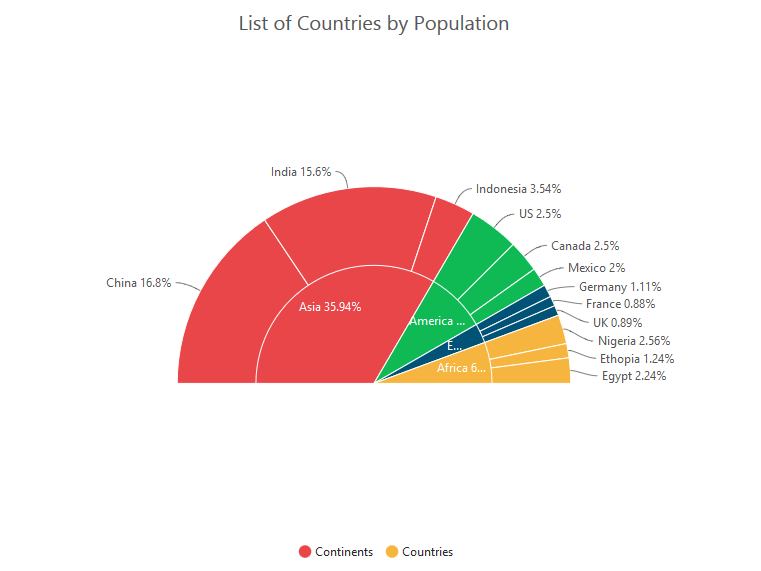Sector of Multiple Doughnut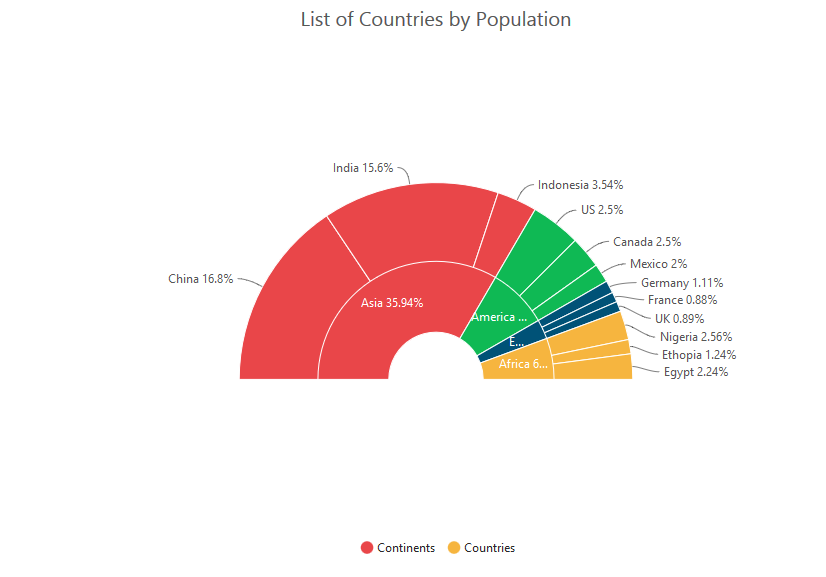## Pyramid Chart

To create a Pyramid chart, you can specify the series Type as Pyramid in the chart series.

• CSHTML
• ``````@(Html.EJ().Chart("chartContainer")

// ...

.Series(sr =>
{
//Set chart type to series
sr.Type(SeriesType.Pyramid)
//...
})

//...
)``````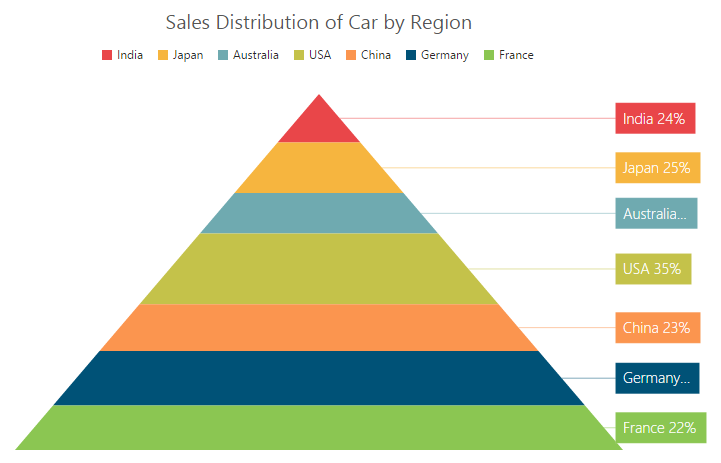### Pyramid Mode

Pyramid mode has two types, Linear and Surface respectively. The default PyramidMode type is “Linear”.

• CSHTML
• ``````@(Html.EJ().Chart("chartContainer")

// ...

.Series(sr =>
{
//Change pyramid mode
sr.PyramidMode(PyramidMode.Surface)
//...
})

//...
)``````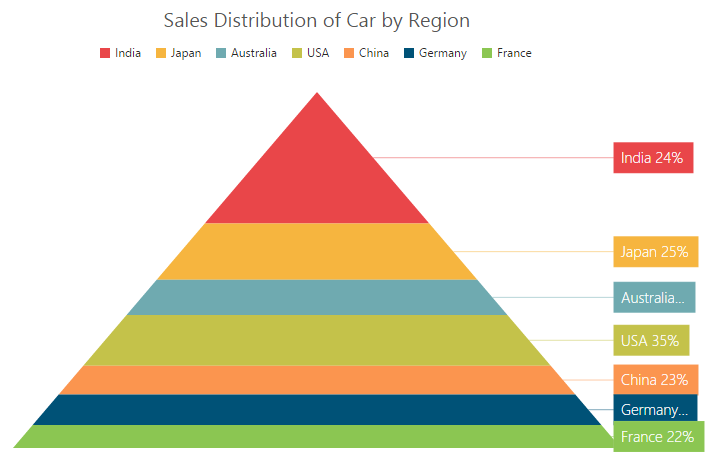### Gap between the segments

You can control the gap between the segments by using the GapRatio option of the series. Its ranges from 0 to 1.

• CSHTML
• ``````@(Html.EJ().Chart("chartContainer")

// ...

.Series(sr =>
{
//Set gapRatio value to pyramid chart
sr.GapRatio(0.1f)
//...
})

//...
)``````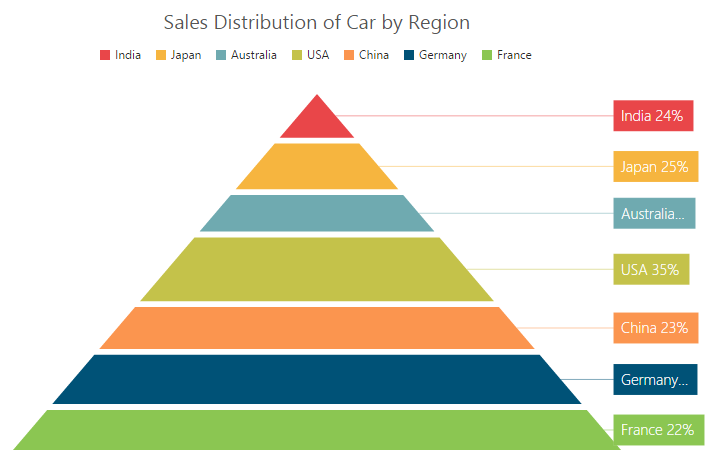### Explode a pyramid segment

You can explode a pyramid segment on the chart load by using the ExplodeIndex of the series.

• CSHTML
• ``````@(Html.EJ().Chart("chartContainer")

// ...

.Series(sr =>
{
//Set point index value to explode the pyramid segment.
sr.ExplodeIndex(4)
//...
})

//...
)``````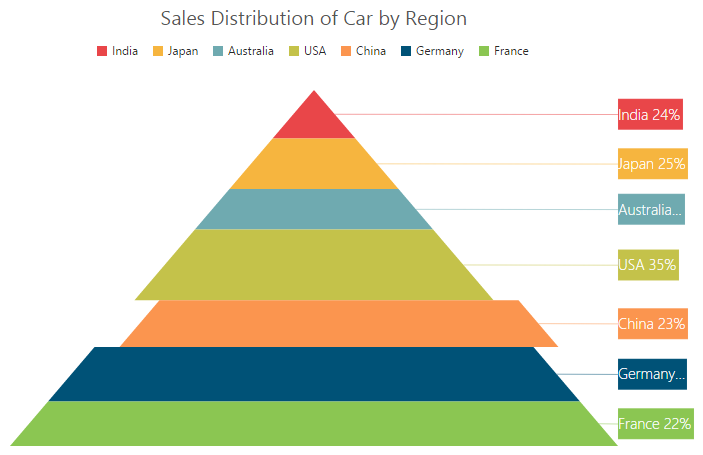## Funnel Chart

You can create a funnel chart by setting the series Type as Funnel in the chart series.

• CSHTML
• ``````@(Html.EJ().Chart("chartContainer")

// ...

.Series(sr =>
{
//Set chart type to series
sr.Type(SeriesType.Funnel)
//...
})

//...
)``````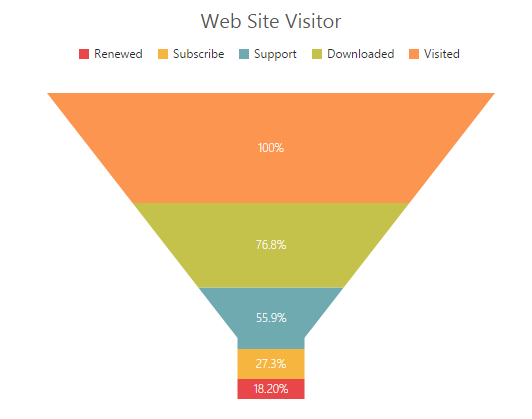### Change the funnel width and height

Funnel segments height and width is calculated from the chart size, by default. You can change this height and width directly without changing the chart size by using the FunnelHeight and FunnelWidth property of the series.

• CSHTML
• ``````@(Html.EJ().Chart("chartContainer")

// ...

.Series(sr =>
{
//Change funnel height and width
sr.FunnelHeight("22%")
.FunnelWidth("25%")
//...
})

//...
)``````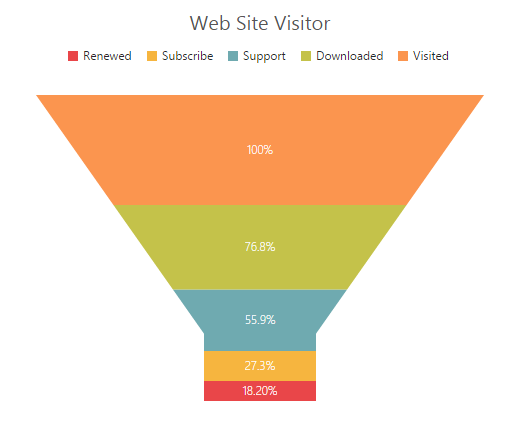### Explode a funnel segment

You can explode a funnel segment on the chart load by using the ExplodeIndex of the series.

• CSHTML
• ``````@(Html.EJ().Chart("chartContainer")

// ...

.Series(sr =>
{
//Set point index value to explode the funnel segment.
sr.ExplodeIndex(3)
//...
})

//...
)``````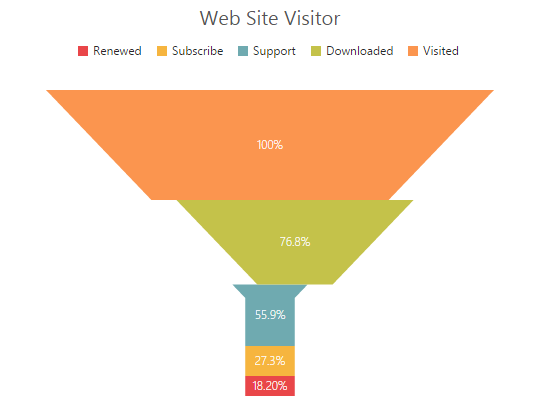## Bubble Chart

To create a Bubble chart, you can set the series Type as Bubble in the chart series. Bubble chart requires 3 fields (X, Y and Size) to plot a point. Here, Size is used to specify the size of each bubble segment.

• CSHARP
• ``````// Create dataSource to bubble chart
List<ChartData> data =new List<ChartData>();
ViewBag.ChartData = data;``````
• CSHTML
• ``````@(Html.EJ().Chart("chartContainer")

// ...

.Series(sr =>
{
//Set chart type to series
sr.Type(SeriesType.Bubble)
//Add datasource and set xName, yName and size to bubble chart
.XName("Month")
.XName("Sales")
.Size("Profit")
.DataSource(ViewBag.ChartData)
//...
})

//...
)``````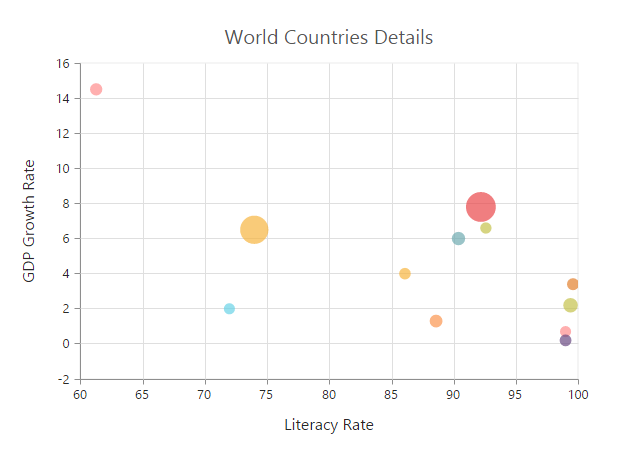## Scatter

To create a Scatter chart, you can set the series Type as Scatter in the chart series.

• CSHTML
• ``````@(Html.EJ().Chart("chartContainer")

// ...

.Series(sr =>
{
//Set chart type to series
sr.Type(SeriesType.Scatter)
//...
})

//...
)``````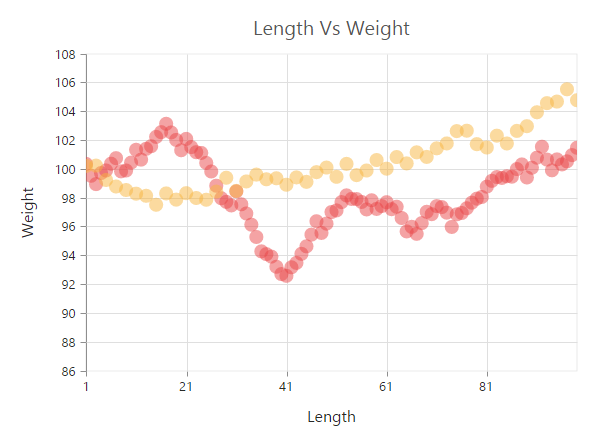### Customize the scatter chart

You can change the scatter size by using the Size property of the series marker. And you can change the scatter color by using the series Fill property.

• CSHTML
• ``````@(Html.EJ().Chart("chartContainer")

// ...

.Series(sr =>
{
sr.Marker(marker=>marker.Size(size=>size.Height(15).Width(15)))
//Set fill color to scatter series
.Fill("#41F282")
//...
})

//...
)``````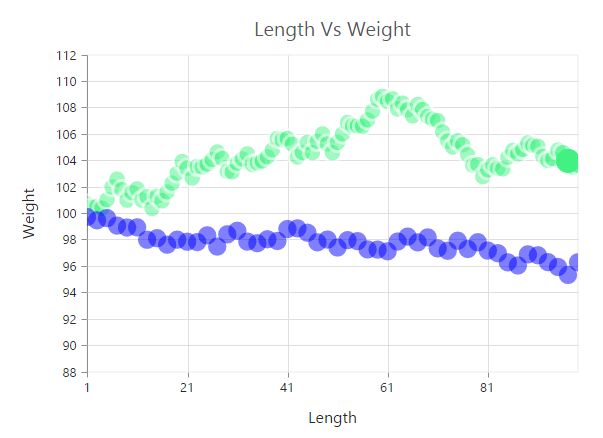## HiLoOpenClose Chart

To create a HiLoOpenClose chart, you can set the series Type as HiloOpenClose in the chart series. HiLoOpenClose chart requires 5 fields (X, High, Low, Open and Close) to plot a segment.

• CSHARP
• ``````// Create dataSource to chart
List<ChartData> data =new List<ChartData>();
data.Add(new ChartData("Feb", 28, 15, 18, 27));
data.Add(new ChartData("Mar", 54, 35, 38, 49));
data.Add(new ChartData("Apr", 52, 21, 35, 29));
ViewBag.ChartData = data;``````
• CSHTML
• ``````@(Html.EJ().Chart("chartContainer")

// ...

.Series(sr =>
{
//Set chart type to series
sr.Type(SeriesType.HiloOpenClose)
//Add datasource and set xName, high and low to HiLo open close chart
.XName("Month")
.High("High")
.Low("Low")
.Open("Open")
.Close("Close")
.DataSource(ViewBag.ChartData)
//...
})

//...
)``````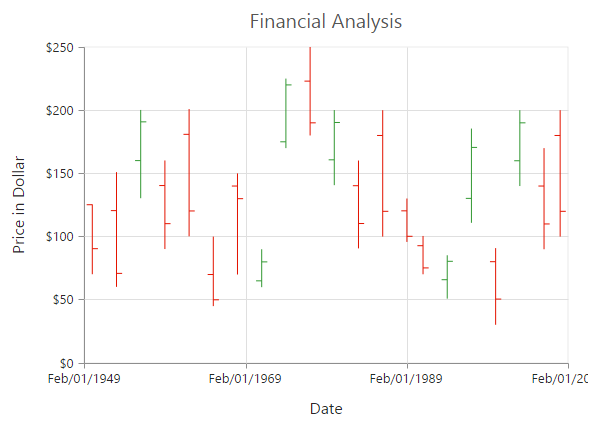### DrawMode

You can change the HiLoOpenClose chart DrawMode to Open, Close or Both. The default value of DrawMode is Both.

• CSHTML
• ``````@(Html.EJ().Chart("chartContainer")

// ...

.Series(sr =>
{
//Change the OHLC drawMode type
sr.DrawMode(SeriesDrawMode.Open)
//...
})

//...
)``````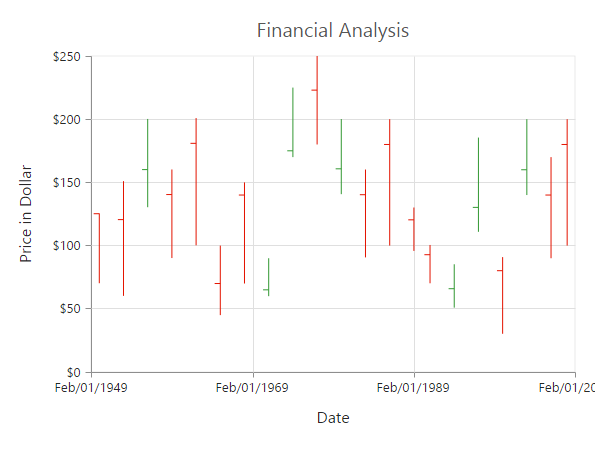### Bull and Bear Color

HiLoOpenClose chart BullFillColor is used to specify a fill color for the segments that indicates an increase in stock price in the measured time interval and BearFillColor is used to specify a fill color for the segments that indicates a decrease in the stock price in the measured time interval.

• CSHTML
• ``````@(Html.EJ().Chart("chartContainer")

// ...

.Series(sr =>
{
//Change bullFill and bearFill color of HiLo open close chart
sr.BearFillColor("#FF6600")
.BullFillColor("#336600")
//...
})

//...
)``````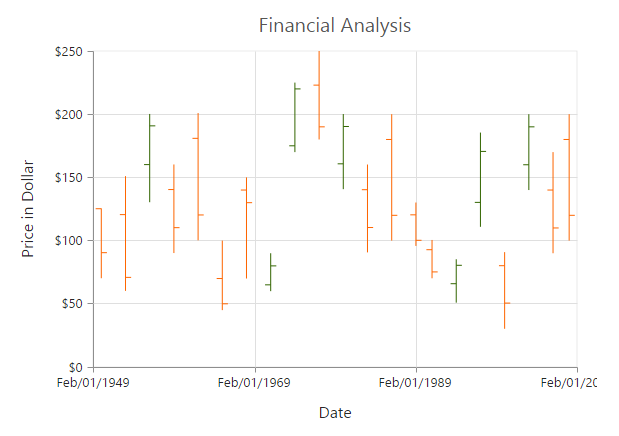## Candle

You can create a Candle chart by specifying the series Type as Candle in the chart series. Candle chart requires 5 fields (X, High, Low, Open and Close) to plot a segment.

• CSHARP
• ``````// Create dataSource to chart
List<ChartData> data =new List<ChartData>();
data.Add(new ChartData("Feb", 28, 15, 18, 27));
data.Add(new ChartData("Mar", 54, 35, 38, 49));
data.Add(new ChartData("Apr", 52, 21, 35, 29));
ViewBag.ChartData = data;``````
• CSHTML
• ``````@(Html.EJ().Chart("chartContainer")

// ...

.Series(sr =>
{
//Set chart type to series
sr.Type(SeriesType.Candle)
//Add datasource and set xName, high and low to candle chart
.XName("Month")
.High("High")
.Low("Low")
.Open("Open")
.Close("Close")
.DataSource(ViewBag.ChartData)
//...
})

//...
)``````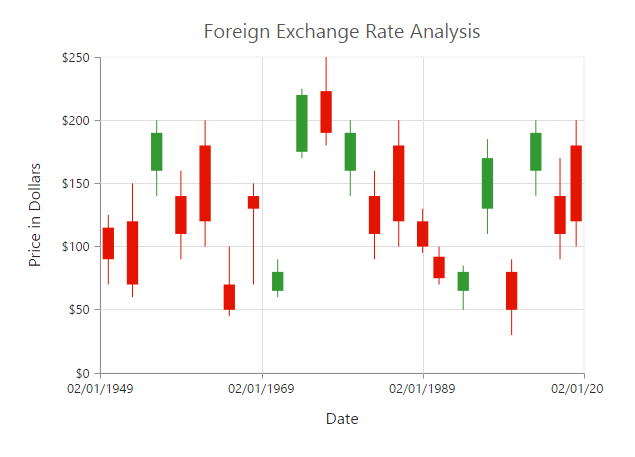### Bull and Bear Color

Candle chart BullFillColor is used to specify a fill color for the segments that indicates an increase in the stock price in the measured time interval and BearFillColor is used to specify a fill color for the segments that indicates a decrease in the stock price in the measured time interval.

• CSHTML
• ``````@(Html.EJ().Chart("chartContainer")

// ...

.Series(sr =>
{
//Change bullFill and bearFill color of candle chart
sr.BearFillColor("#FF6600")
.BullFillColor("#336600")
//...
})

//...
)``````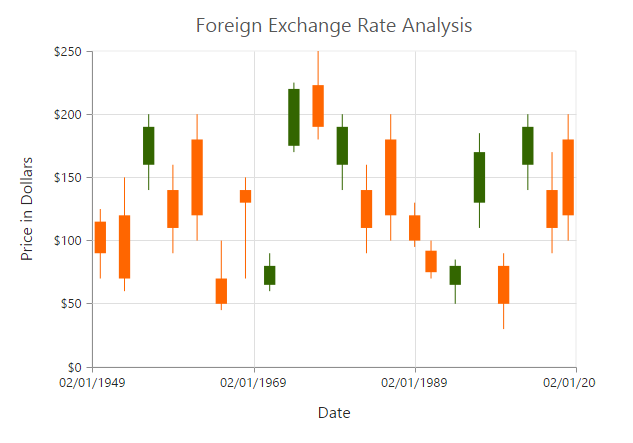## HiLo

HiLo chart is created by setting the series Type as Hilo in the chart series. HiLo chart requires 3 fields (X, High and Low) to plot a segment.

• CSHARP
• ``````// Create dataSource to chart
List<ChartData> data =new List<ChartData>();
ViewBag.ChartData = data;``````
• CSHTML
• ``````@(Html.EJ().Chart("chartContainer")

// ...

.Series(sr =>
{
//Set chart type to series
sr.Type(SeriesType.Hilo)
//Add datasource and set xName, high and low to HiLo chart
.XName("Month")
.High("High")
.Low("Low")
//...
})

//...
)``````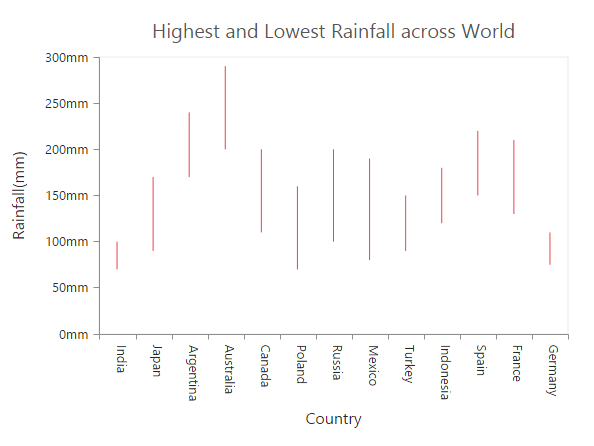## Polar

Polar chart is created by setting the series Type as Polar in the chart series.

• CSHTML
• ``````@(Html.EJ().Chart("chartContainer")

// ...

.Series(sr =>
{
//Set chart type to series
sr.Type(SeriesType.Polar)
//...
})

//...
)``````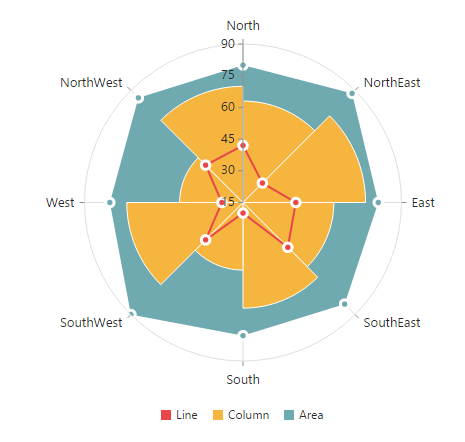### DrawType

Polar DrawType property is used to change the series plotting type to Line, Column or Area. The default value of DrawType is Line.

• CSHTML
• ``````@(Html.EJ().Chart("chartContainer")

// ...

.Series(sr =>
{
//Change polar series drawType
sr.DrawType(DrawType.Column)
//...
})

//...
)``````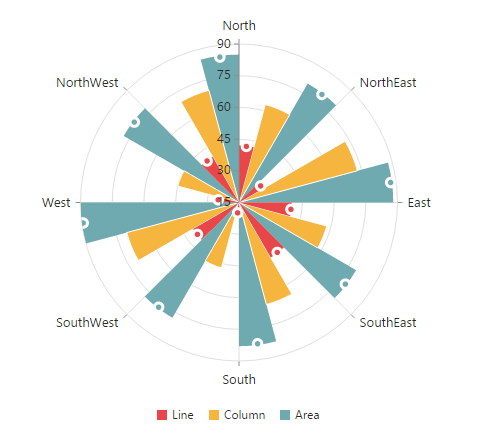### Stack columns in Polar chart

By using the IsStacking property, you can specify whether the column has to be stacked when the DrawType is column. Its default value is false.

• CSHTML
• ``````@(Html.EJ().Chart("chartContainer")

// ...

.Series(sr =>
{
//Enable isStacking property for stacked column polar chart
sr.IsStacking(true)
//...
})

//...
)``````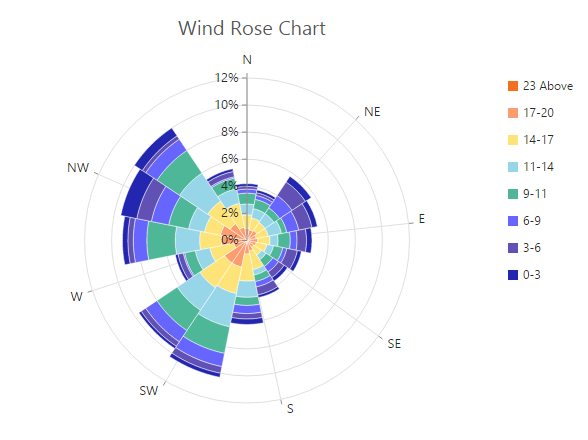Click here to view the Polar Wind Rose Chart online demo sample.

To create a Radar chart, you can specify the series Type as Radar in the chart series.

• CSHTML
• ``````@(Html.EJ().Chart("chartContainer")

// ...

.Series(sr =>
{
//Set chart type to series
//...
})

//...
)``````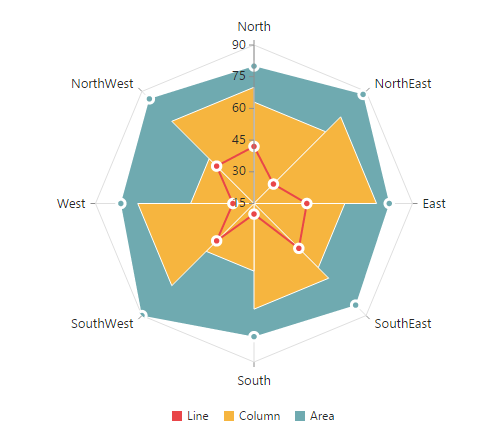### DrawType

Radar DrawType property is used to change the series plotting type to Line, Column or Area. The default value of DrawType is Line.

• CSHTML
• ``````@(Html.EJ().Chart("chartContainer")

// ...

.Series(sr =>
{
sr.DrawType(DrawType.Column)
//...
})

//...
)``````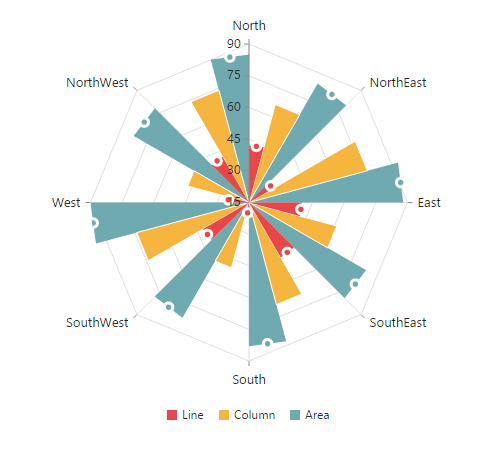### Stack columns in Radar chart

By using the IsStacking property, you can specify whether the column has to be stacked when the DrawType is set as Column. Its default value is set to false.

• CSHTML
• ``````@(Html.EJ().Chart("chartContainer")

// ...

.Series(sr =>
{
//Enable isStacking property for stacked column radar chart
sr.IsStacking(true)
//...
})

//...
)``````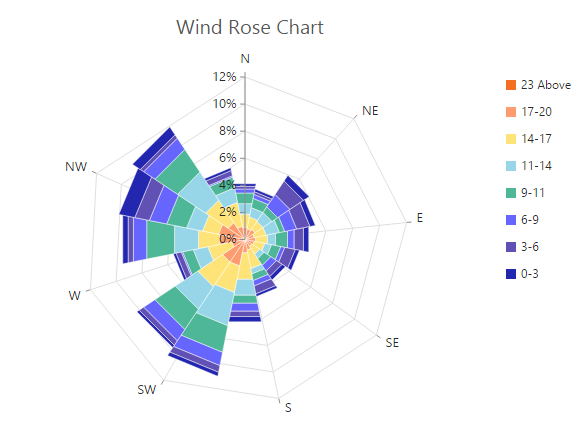## Waterfall Chart

For rendering a Waterfall chart, set series Type as Waterfall in the chart series. To change the waterfall series segment color use Fill option of series and use PositiveFill property to differentiate the positive segments.

NOTE

The inline property of the Series.PositiveFill has the first priority and override the Series.Fill.

• CSHTML
• ``````@(Html.EJ().Chart("container")

.Series(sr =>
{
//...
//Change series type
sr.Type(SeriesType.Waterfall)
})
//...
)``````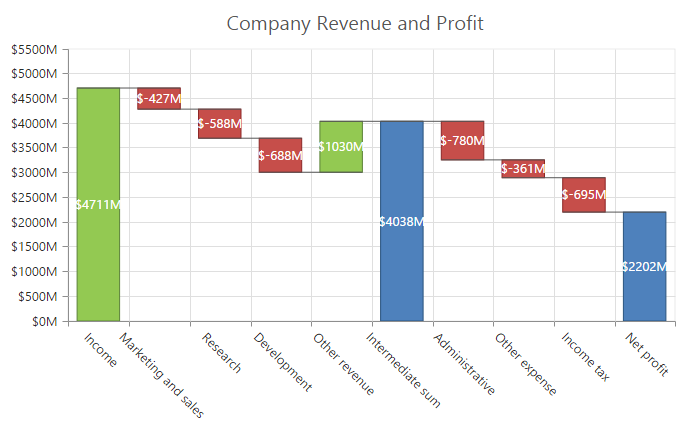ShowIntermediateSum

To display the summary of values since the last intermediate point of the waterfall series, set ShowIntermediateSum property as true in the specific point.

• CSHTML
• ``````@(Html.EJ().Chart("container")

//...
.Series(sr =>
{
sr.Points(pt =>
{
//Enable showIntermediateSum in to a point.
//...

})

//...
)``````

ShowTotalSum

The sum of all previous point in the waterfall series is displayed on enabling the ShowTotalSum property for a specific point.

• CSHTML
• ``````@(Html.EJ().Chart("container")

//...
.Series(sr =>
{
sr.Points(pt =>
{
//Enable showTotalSum in to a point.
//...

})

//...
)``````

### ConnectorLine

To customize the connector line color, width, opacity and dashArray of the waterfall series, you can use ConnectorLine option of series.

• CSHTML
• ``````@(Html.EJ().Chart("container")
.Series(sr =>
{
sr .ConnectorLine(cl=>cl.Color("#333000")
.Width(1).Opacity(1).DashArray("2,3"))
})

//...
)``````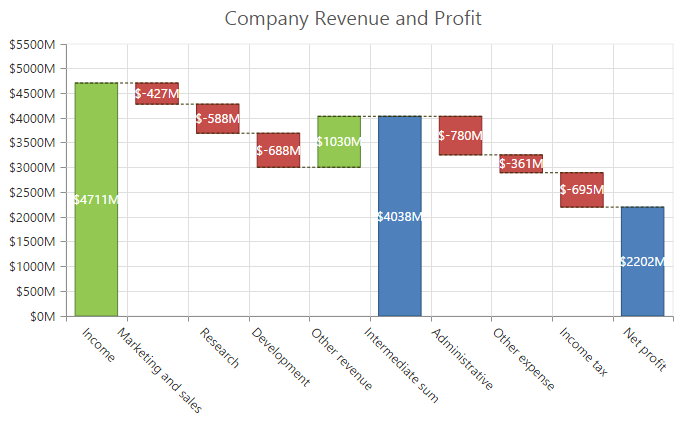## ErrorBar Chart

EjChart can generate Error bar for Cartesian type series (Line, Column, Bar, Scatter, Area, Candle, HiLo, etc.). To render the Error bar for the series, set Visibility as “visible” to ErrorBar in the series.

• CSHTML
• ``````@(Html.EJ().Chart("container")

//...
.Series(sr =>
{
//To toggle the error bar visibility
sr.ErrorBar(era =>

})
//...
)``````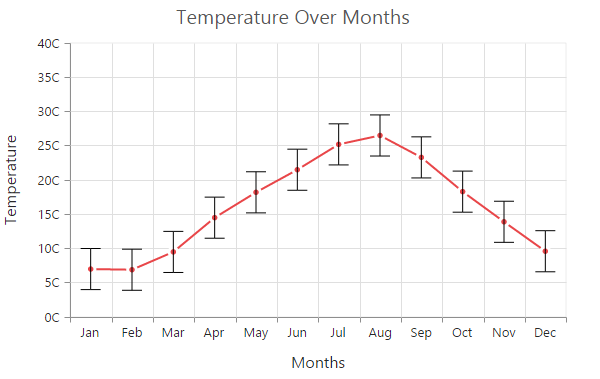### Changing Error Bar Type

You can change the error bar rendering type using Type (like FixedValue, Percentage, StandardDeviation, StandardError and Custom) option of errorBar. To change the error bar line length you can use VerticalErrorValue property.

• CSHTML
• ``````@(Html.EJ().Chart("container")

//...
.Series(sr =>
{
//To change the error bar type
sr.ErrorBar(era =>
era.Type(ErrorBarType.FixedValue)
})
//...
)``````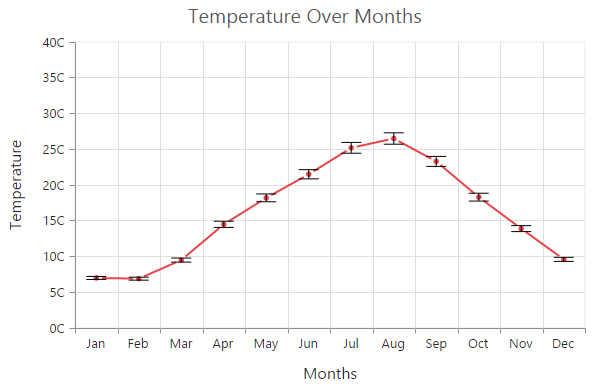#### Customizing error bar type

To customize the error bar type, set error bar Type as Custom and then change the horizontal/vertical positive and negative value of error bar.

• CSHTML
• ``````@(Html.EJ().Chart("container")

//...
.Series(sr =>
{
//To change the error bar type.
sr.ErrorBar(era =>
era.Type(ErrorBarType.Custom)
.VerticalPositiveErrorValue(5)
.HorizontalPositiveErrorValue(1)
.VerticalNegativeErrorValue(5)
})
//...
)``````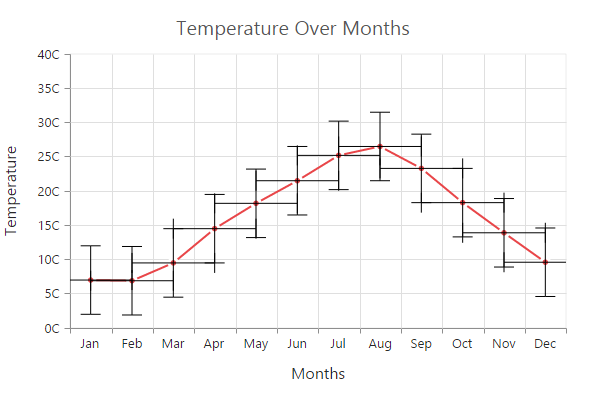### Changing Error Bar Mode

Error bar mode is used to define whether the error bar line has to be drawn Horizontally, Vertically or in Both side. To change the error bar mode use ErrorBar.Mode option.

• CSHTML
• ``````@(Html.EJ().Chart("container")
//...
.Series(sr =>
{
//To change the error bar mode.
sr.ErrorBar(era =>
era.Type(ErrorBarType.FixedValue)
})
//...
)``````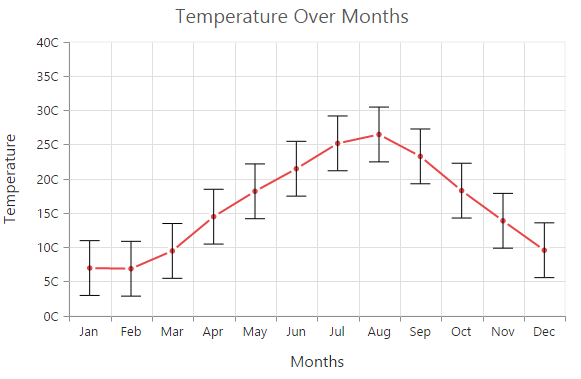### Changing Error Bar Direction

You can change the error bar direction to plus, minus or both side using ErrorBar.Directions option.

• CSHTML
• ``````@(Html.EJ().Chart("container")
//...
.Series(sr =>
{
//To change the error bar direction
sr.ErrorBar(era =>
era.Type(ErrorBarType.FixedValue)
.Mode(ErrorBarMode.Vertical)
})
//...
)``````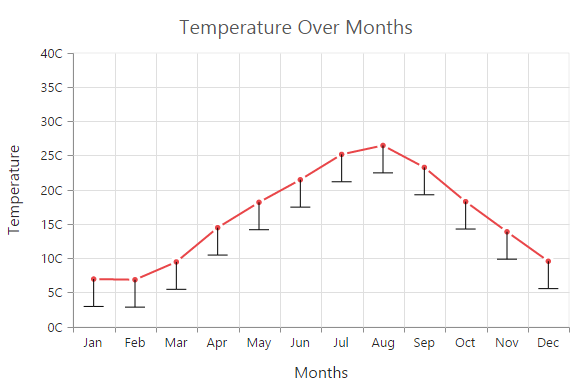### Customizing Error bar cap

To customize the errorBar cap visibility, length, width and fill color, you can use Cap option in the Series.ErrorBar.

• CSHTML
• ``````@(Html.EJ().Chart("container")

//...
.Series(sr =>
{
//To customize the error bar cap
sr.ErrorBar(era =>
era.Cap(cap=>
cap.Visible(true)
.Length((20),
.Width(1),
})
//...
)``````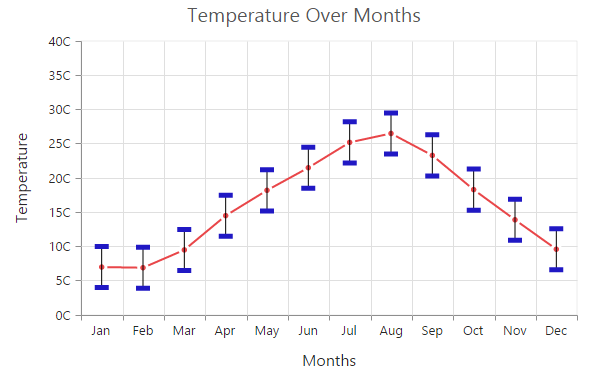## Box and Whisker Chart

To render a Box and Whisker Chart, set the series type as BoxAndWhisker .Box and Whisker chart requires
2 fields (x and y) to plot a segment. The field y requires n number of data or it should contain minimum of five values to plot a segment.

• CSHTML
• ``````@(Html.EJ().Chart("container")

//...
.Series(sr =>
{
sr.Points(pt =>
{
})
.Type(SeriesType.BoxAndWhisker)
})
//...
)``````### BoxPlotMode

You can change the rendering mode of the Box and Whisker series using the BoxPlotMode property .The default value of BoxPlotMode is exclusive.
The other boxPlotModes available are `inclusive` and `normal`.

• CSHTML
• ``````@(Html.EJ().Chart("container")

//...
.Series(sr =>
{
.BoxPlotMode(BoxPlotMode.Inclusive)
})
//...
)``````

### ShowMedian

Box and Whisker showMedian property is used to show the box and whisker average value. The default value of showMedian is false.

• CSHTML
• ``````@(Html.EJ().Chart("container")

//...
.Series(sr =>
{
.ShowMedian(true)
})
//...
)``````### Customize the Outlier

Outlier symbol, width and height can be customized using outlierSettings through outlierSettings property. By default Outlier symbol is displayed as circle with a height and width of 6 pixels.

• CSHTML
• ``````@(Html.EJ().Chart("container")

//...
.Series(sr =>
{
.OutlierSettings(outlier=>outlier.Shape(ChartShape.Triangle)
})
//...
)``````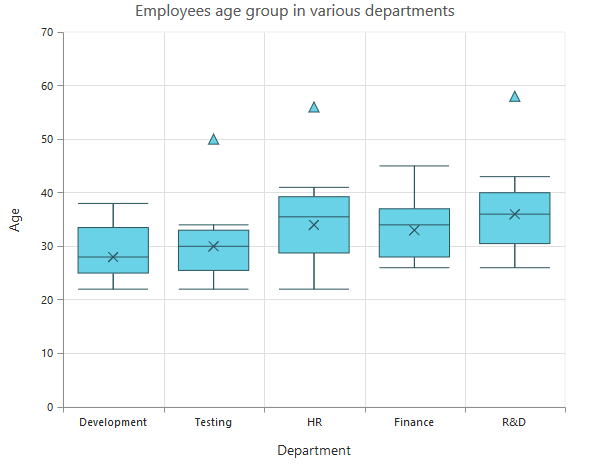Click here to view the Box and Whisker Chart online demo sample.

## Pie Of Pie Chart

To render the pie of pie chart, set the series `Type` as PieOfPie. Pie of pie chart is used for displaying the data of a pie slice as another pie chart. The values in the second pie is displayed based on the splitMode property.

• CSHTML
• ``````@(Html.EJ().Chart("container")
.Series(sr =>
{
sr.Points(pt =>
{
})
.SplitValue("10")
})

)``````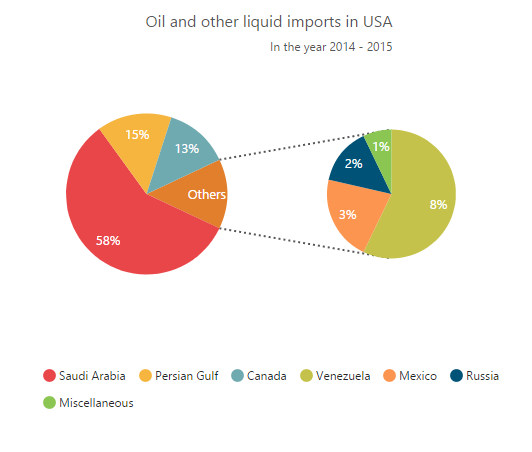Click here to view the Pie Of Pie Chart online demo sample.

### Split Mode and Split Value

The points to be displayed in the second pie is decided based on the `splitMode` property.SplitMode property takes the following values.

• Position – Have to split the data points based on its position
• Value – Have to split the data points based on its Y value
• Percentage – Have to split the points based on the percentage value
• Indexes – The data points with the specified indexes are split separately
By default, the splitMode is set to Value.
• CSHTML
• ``````@(Html.EJ().Chart("container")

//...
.Series(sr =>
{
.SplitMode(SplitMode.Position)
})
//...
)``````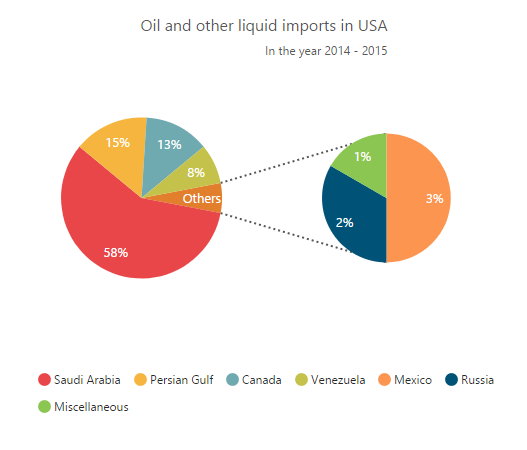### Changing Pie Of Pie Size

The size of the second Pie can be customized by using the `PieOfPieCoefficient` property. The default value of pieOfPieCoefficient is 0.6.Its value ranges from 0 to 1.

• CSHTML
• ``````@(Html.EJ().Chart("container")

//...
.Series(sr =>
{
})
//...
)``````

The following screenshot represents the pie of pie series with pieOfPieCoefficient as 1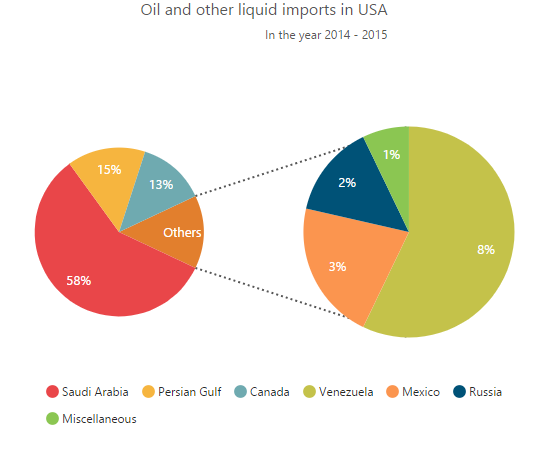#### Customizing the Gap

The distance between the two pies in the pie of pie chart can be controlled by using the `GapWidth` property. The default value is 50.

• CSHTML
• ``````@(Html.EJ().Chart("container")

//...
.Series(sr =>
{